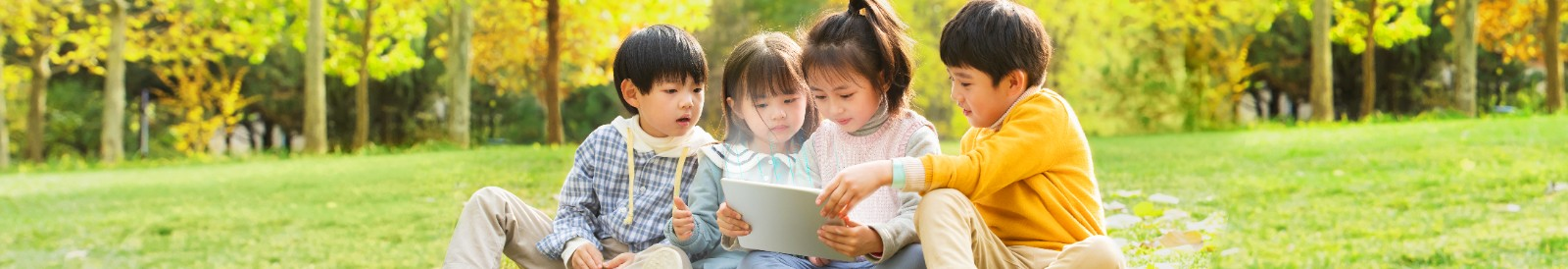•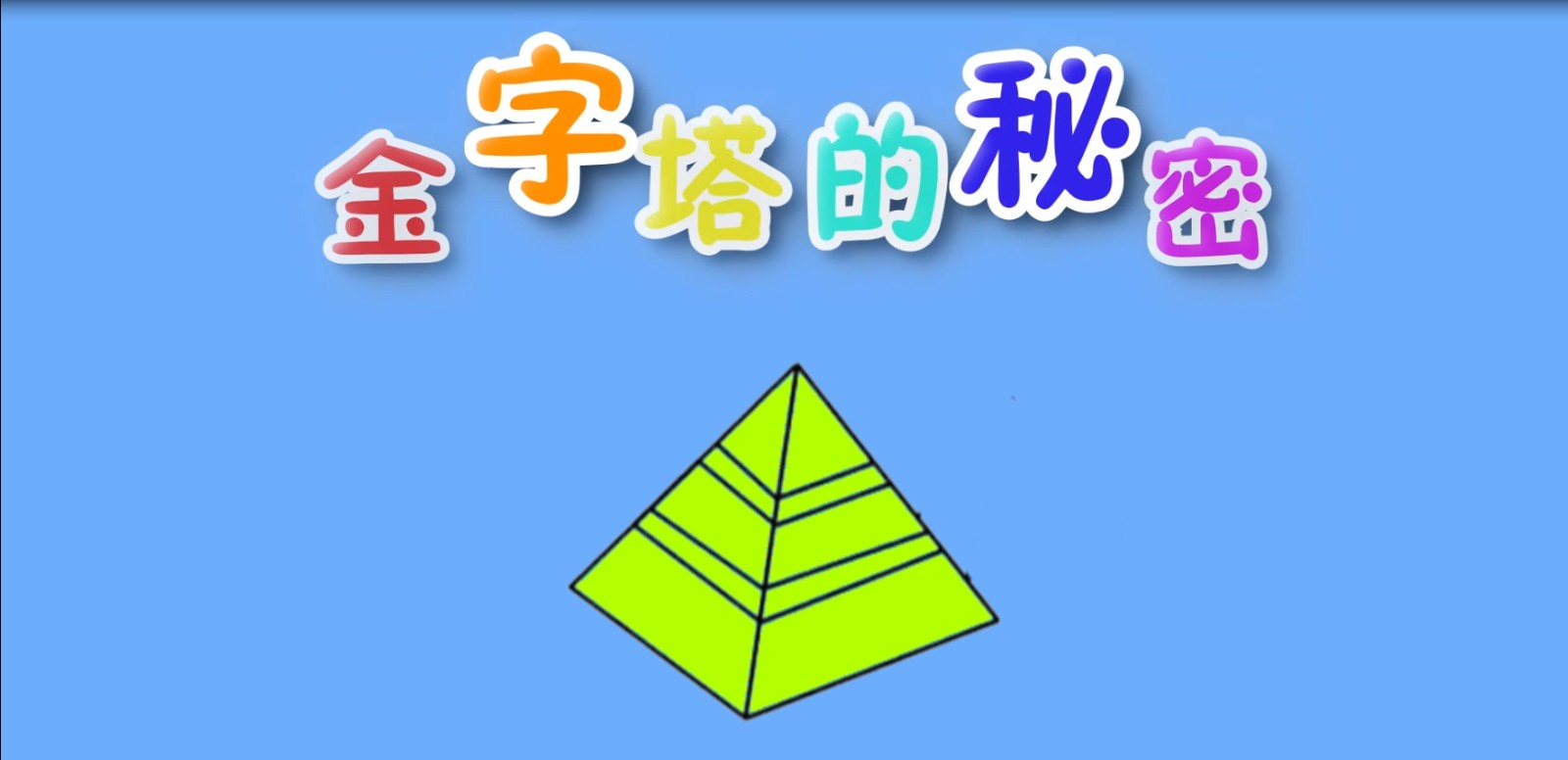金字塔的秘密
•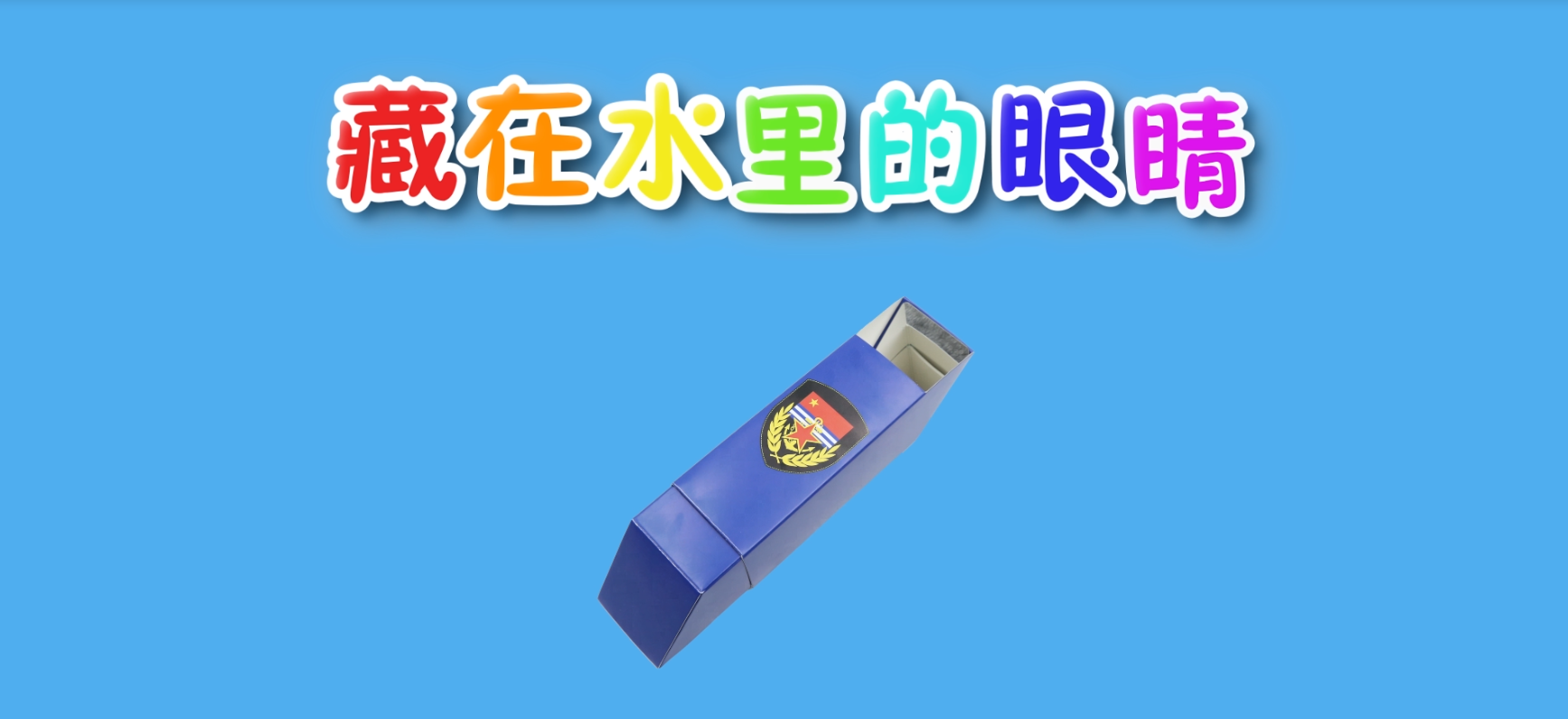藏在水里的眼睛
•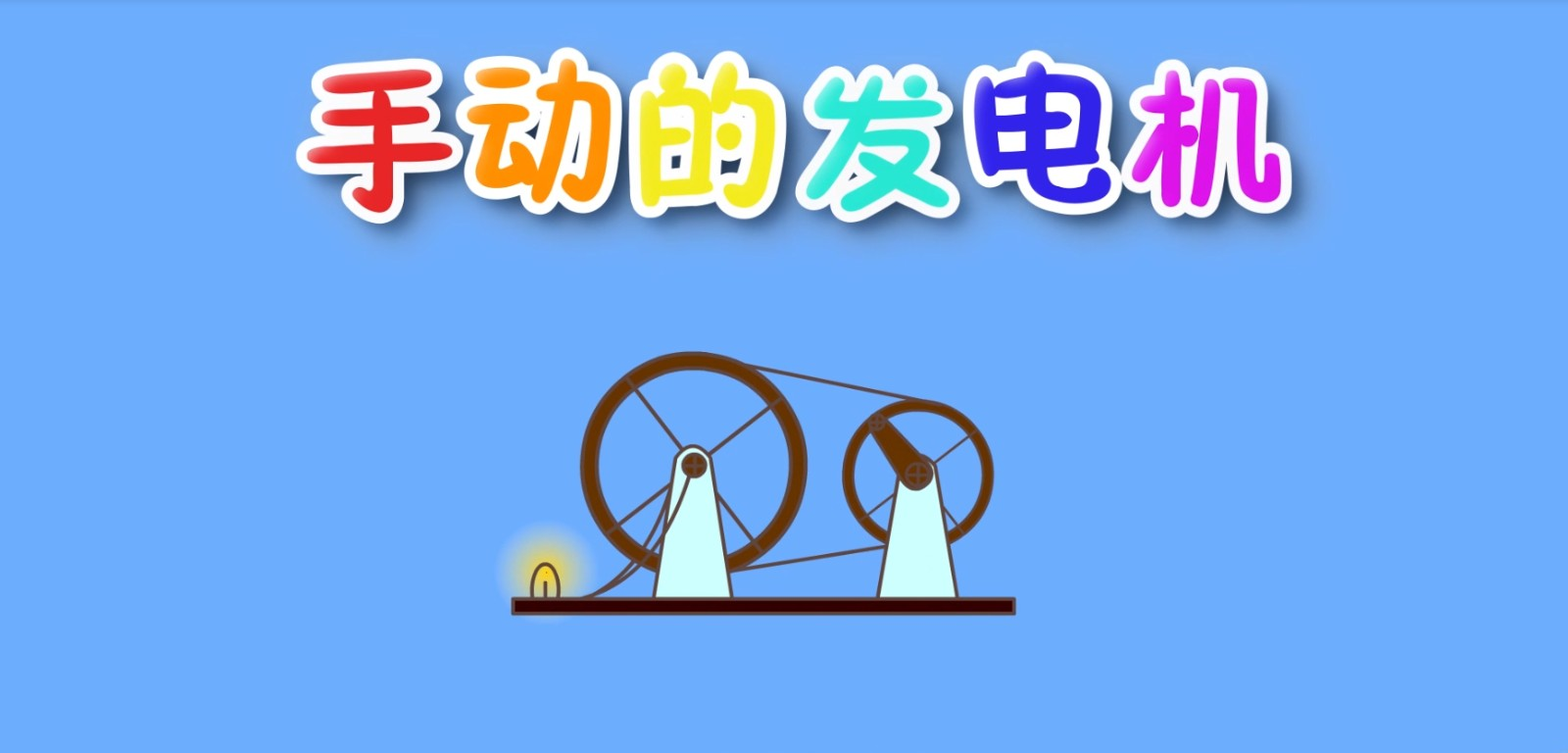手动的发电机
•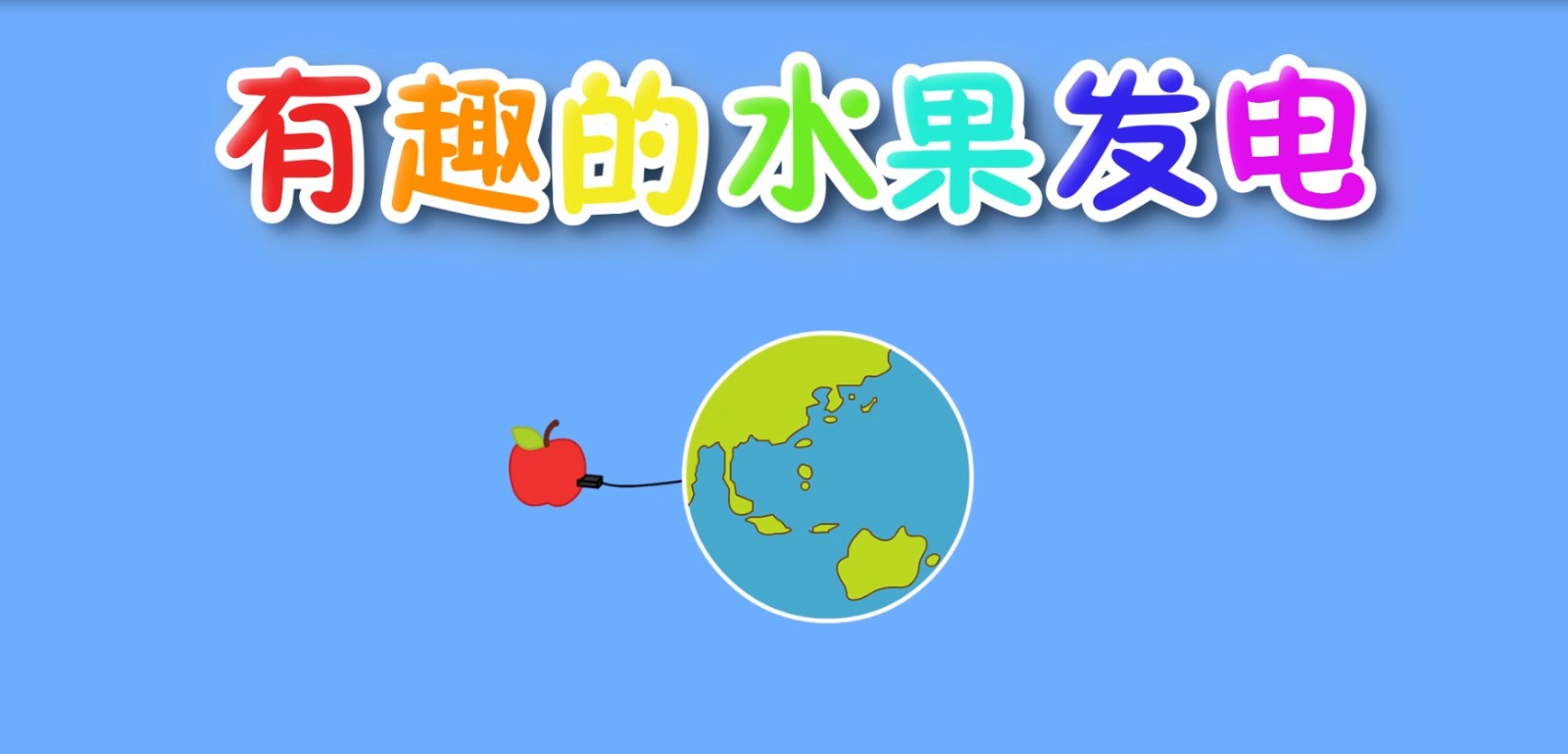有趣的水果发电

•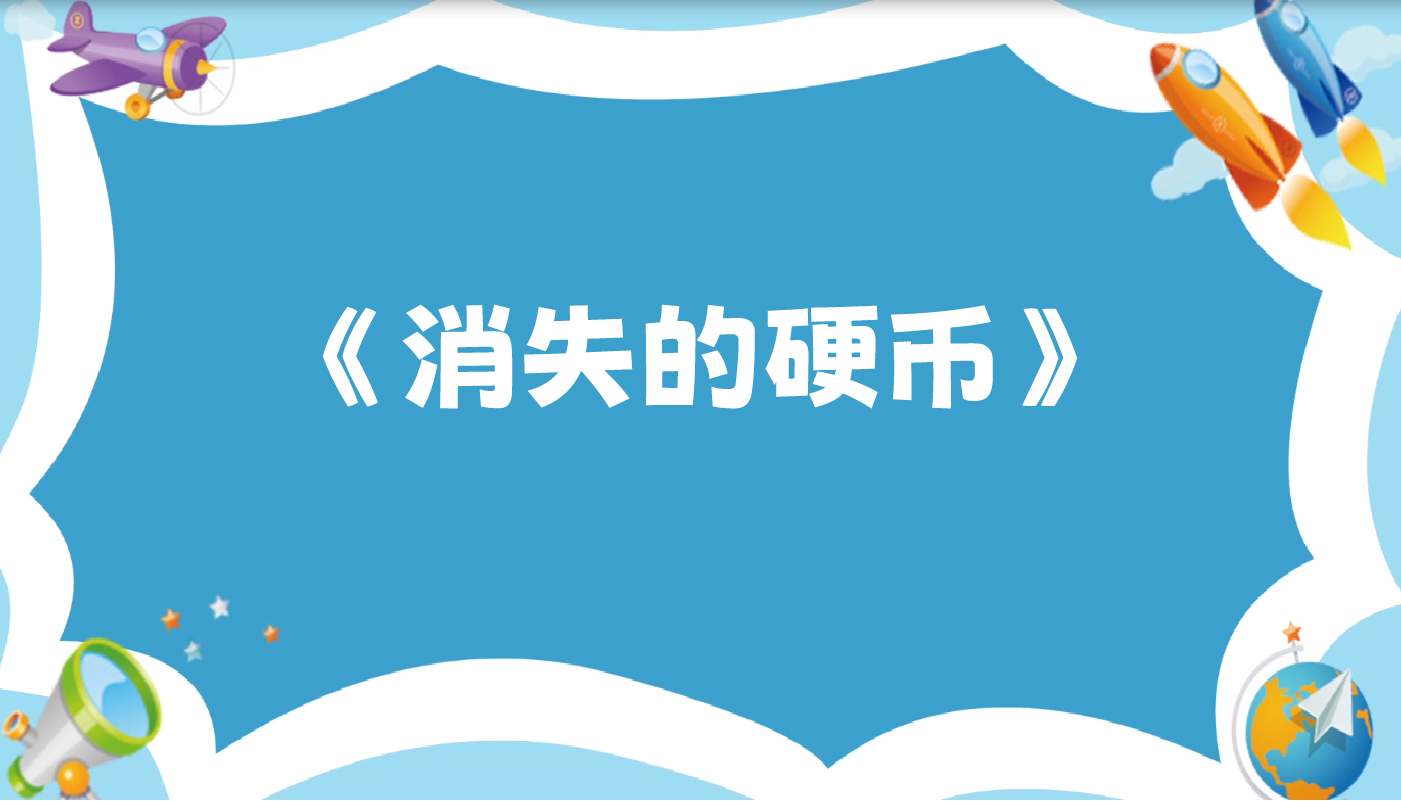消失的硬币
•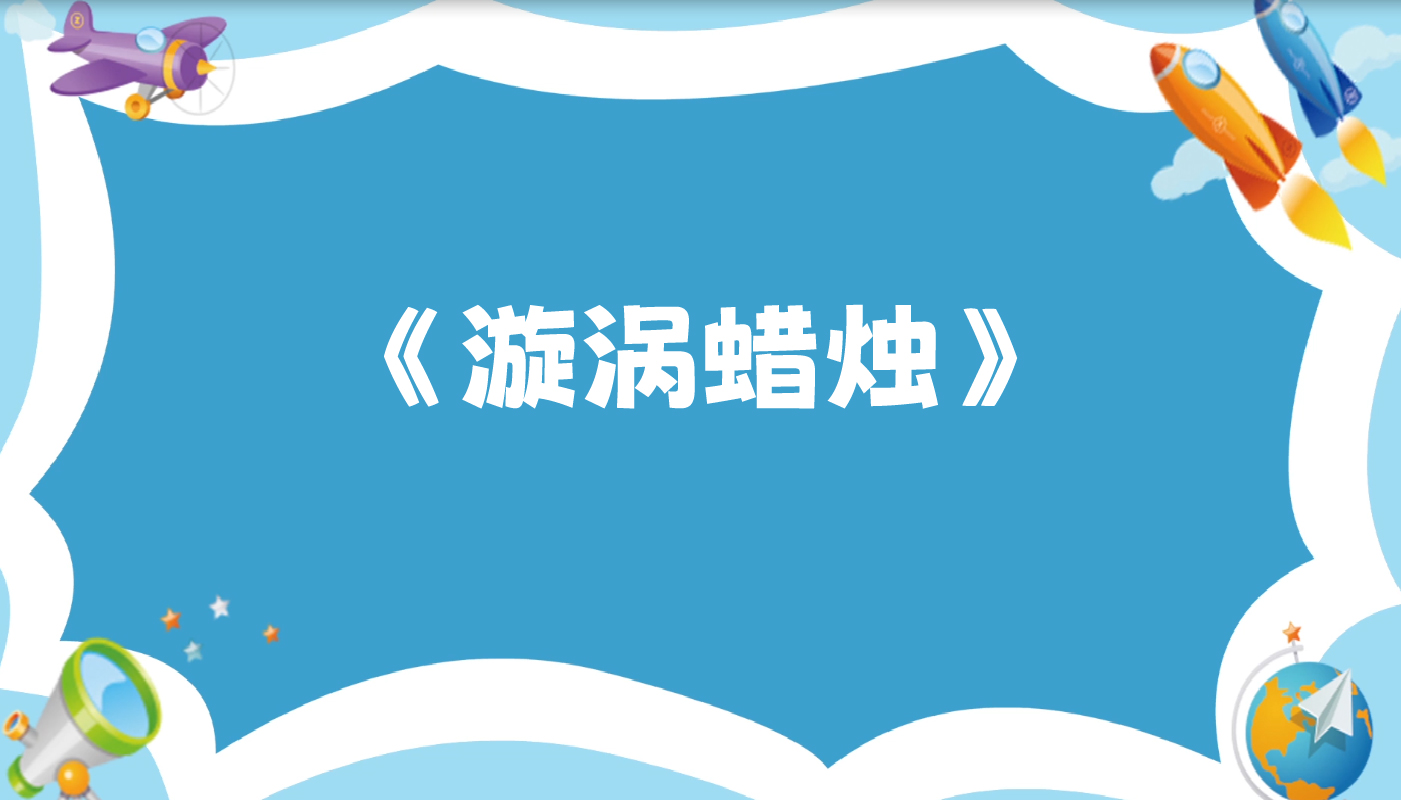漩涡蜡烛
•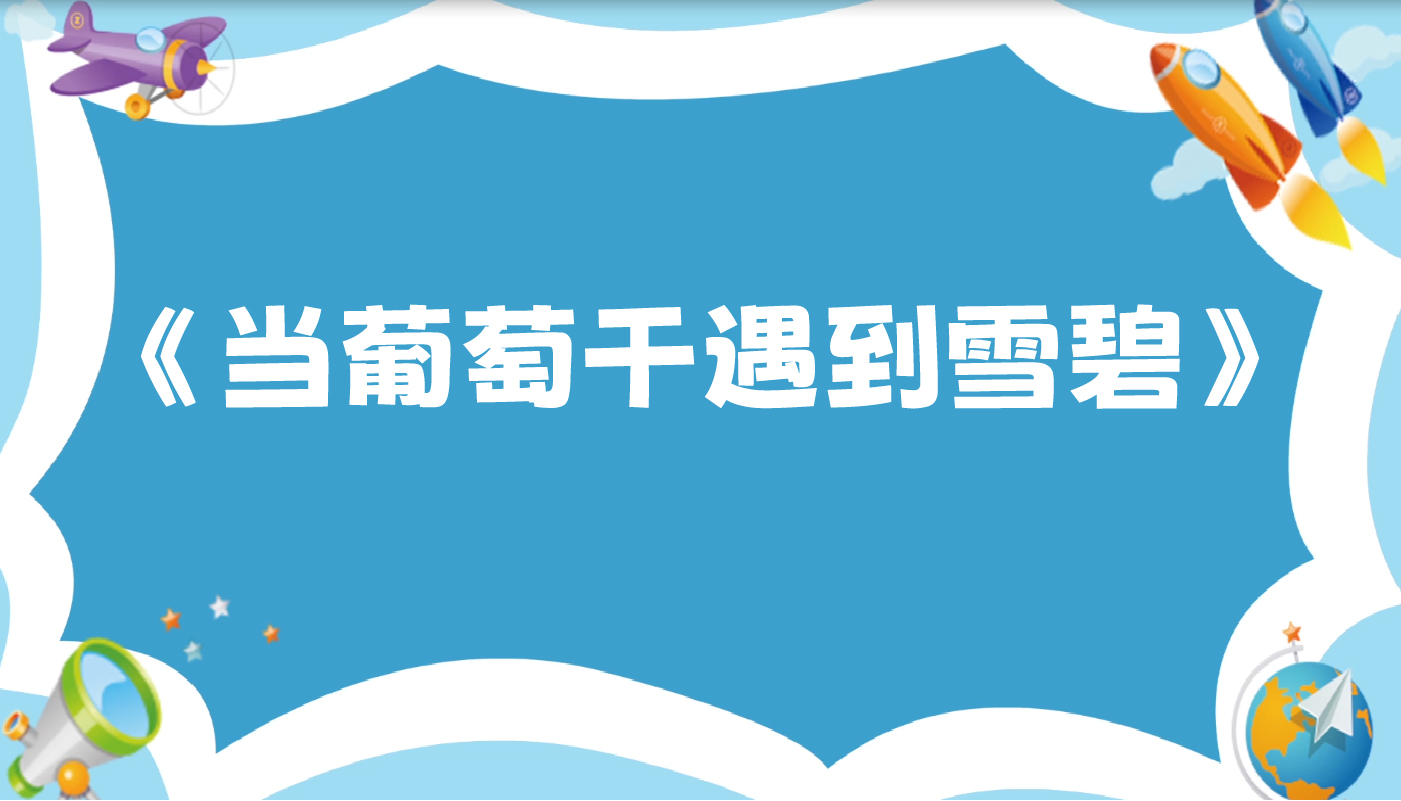当葡萄干遇到雪碧
•漂浮的鸡蛋

•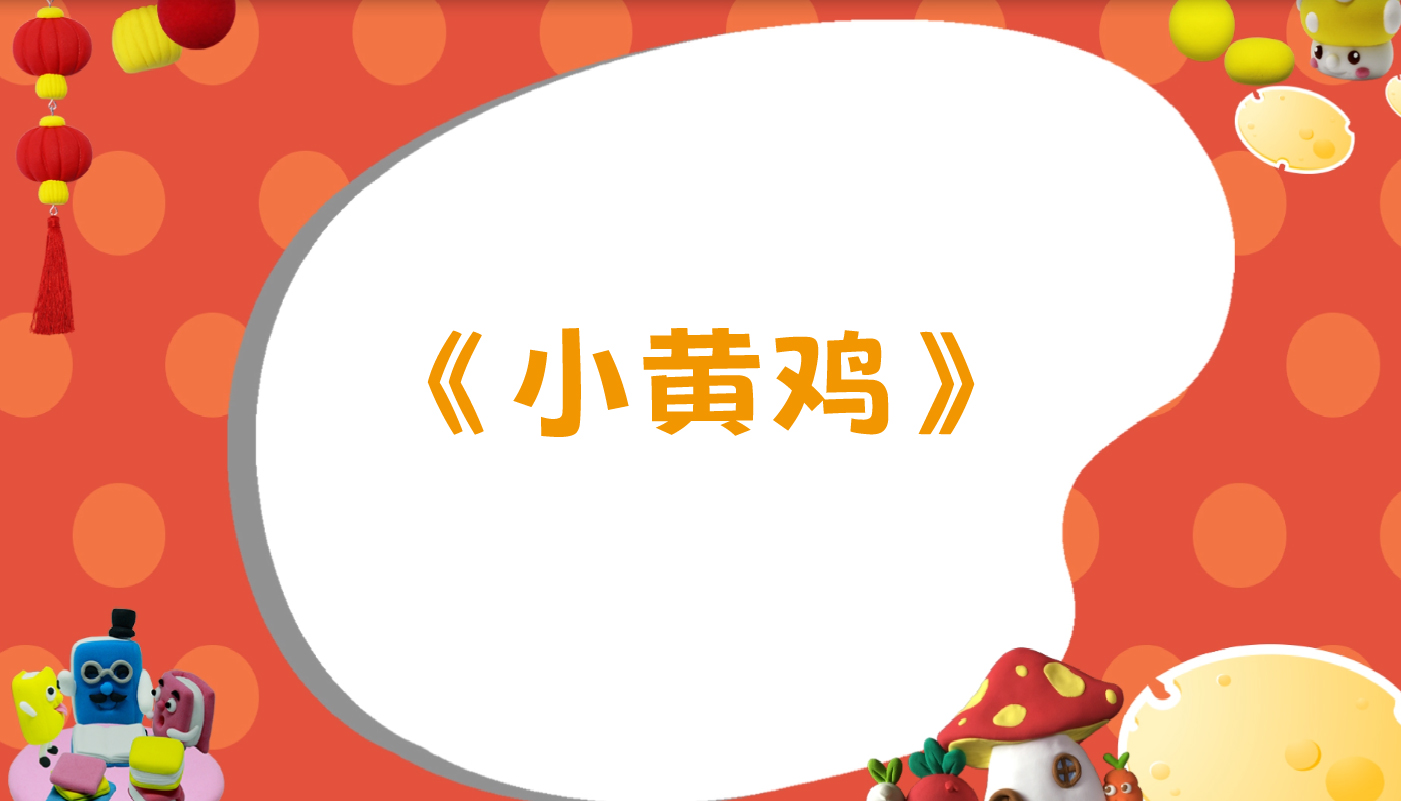小黄鸡
•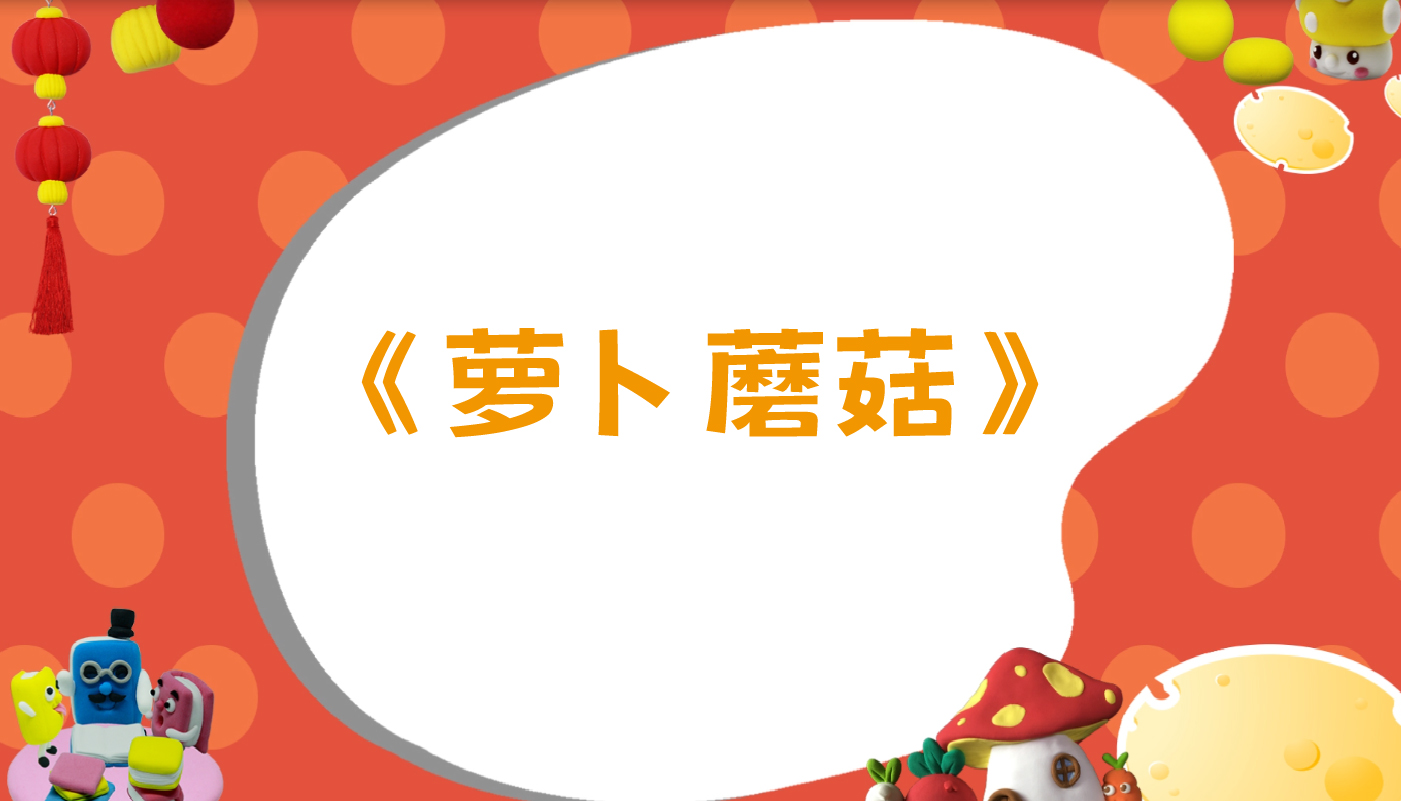萝卜蘑菇
•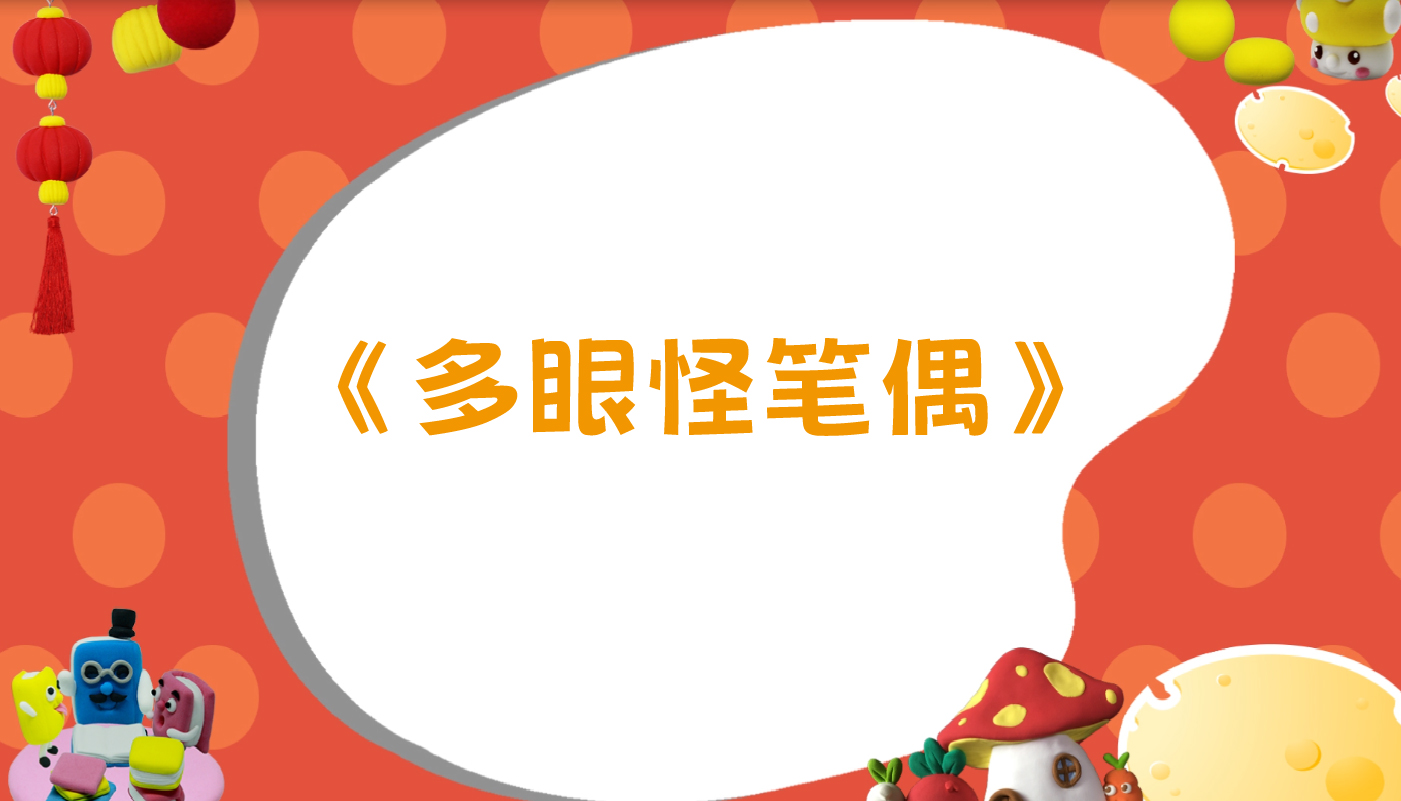多眼怪笔偶
•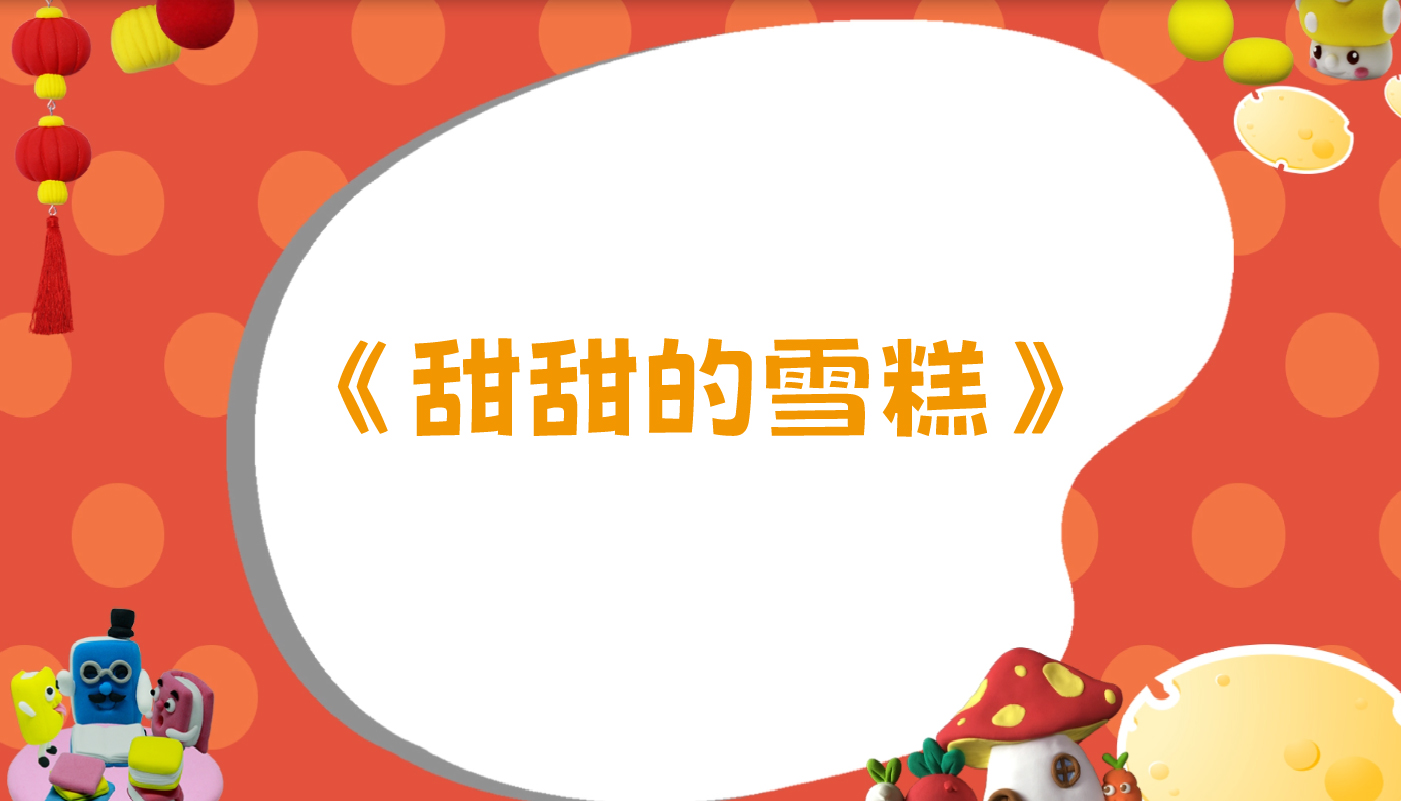甜甜的雪糕

•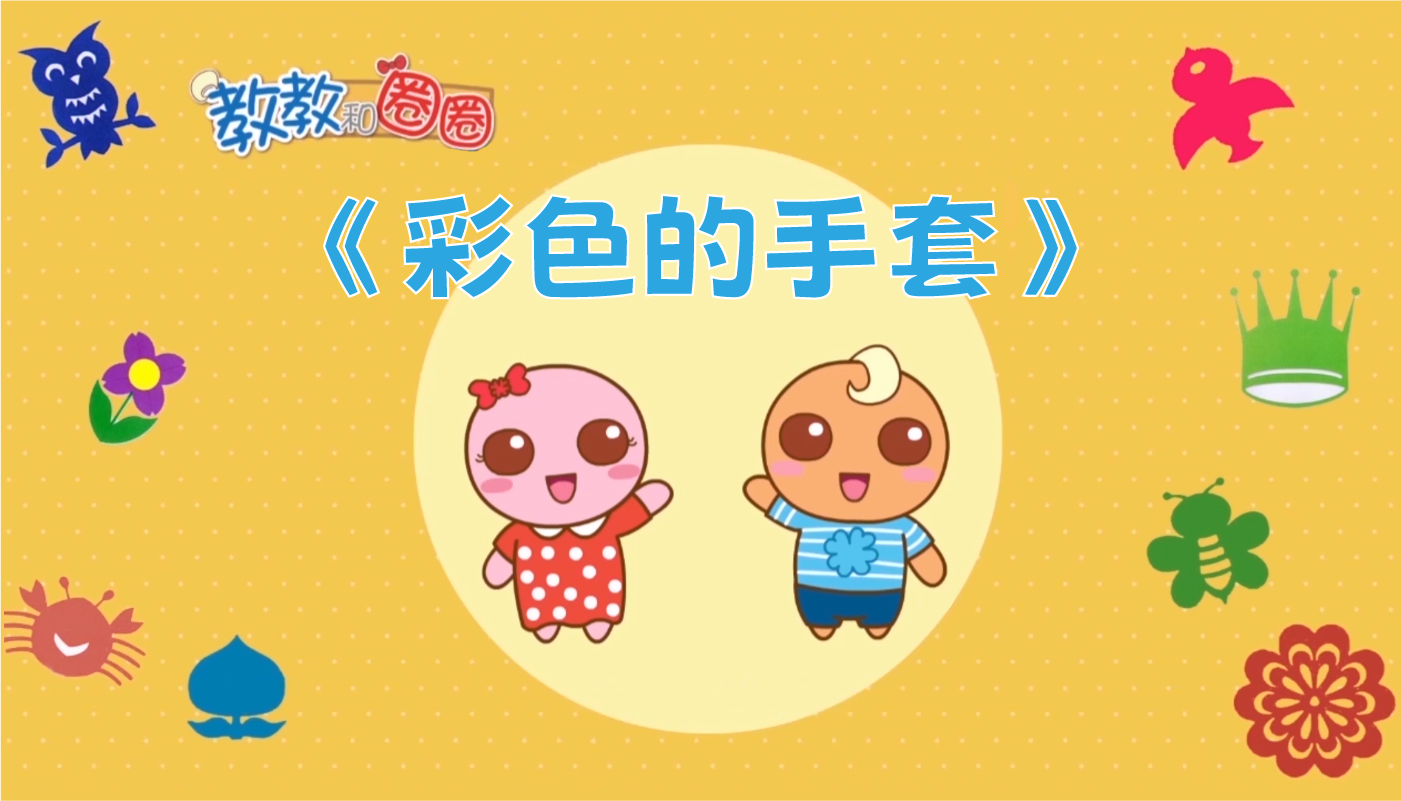彩色的手套
•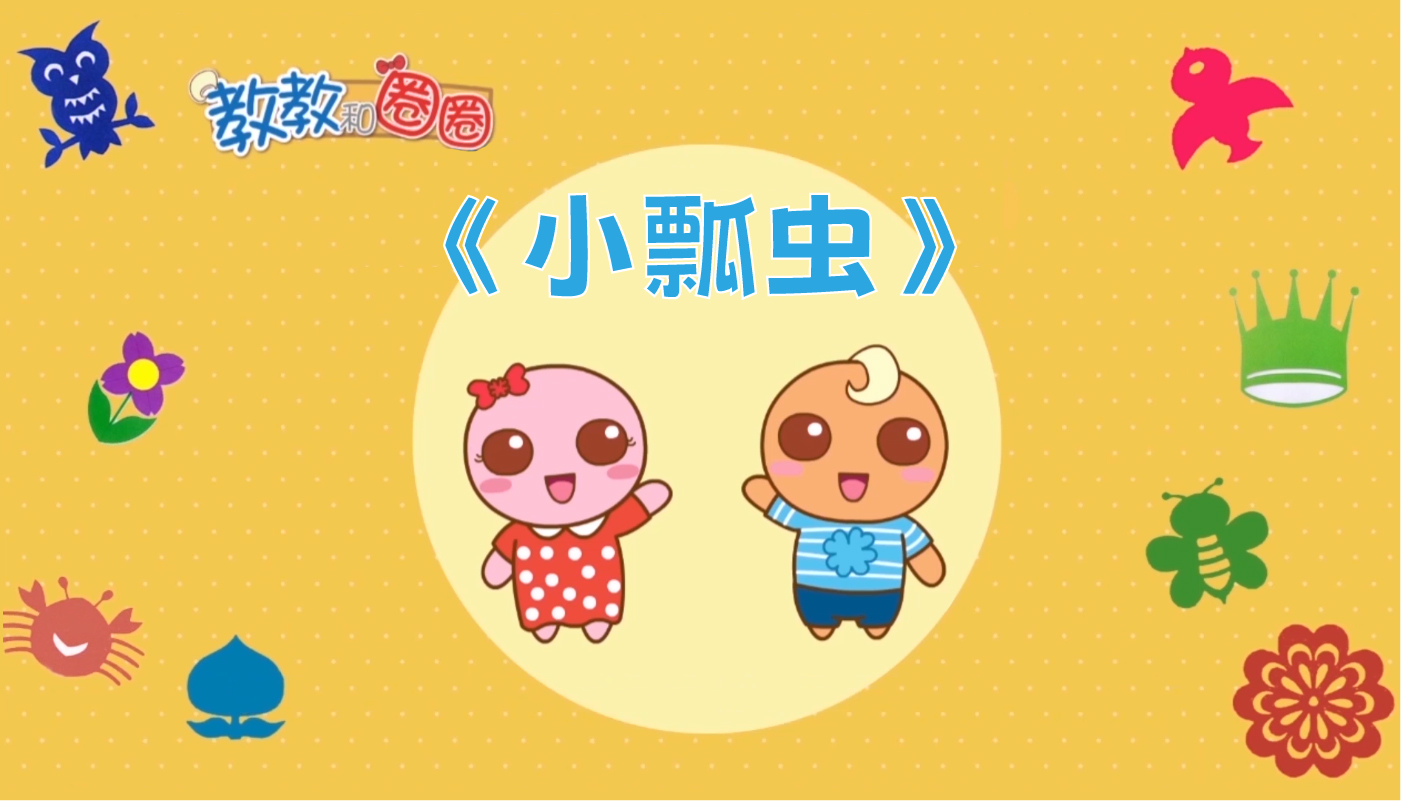小瓢虫
•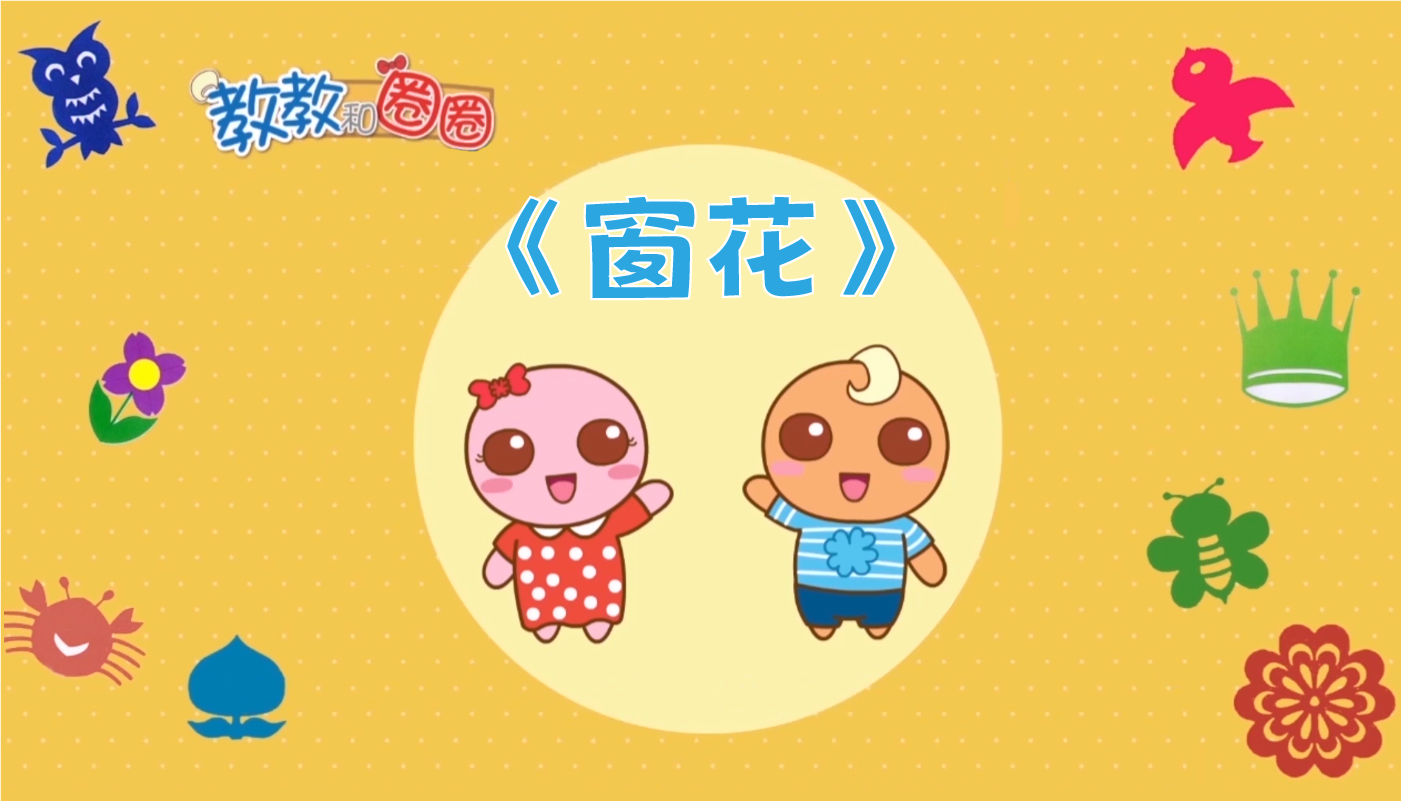窗花
•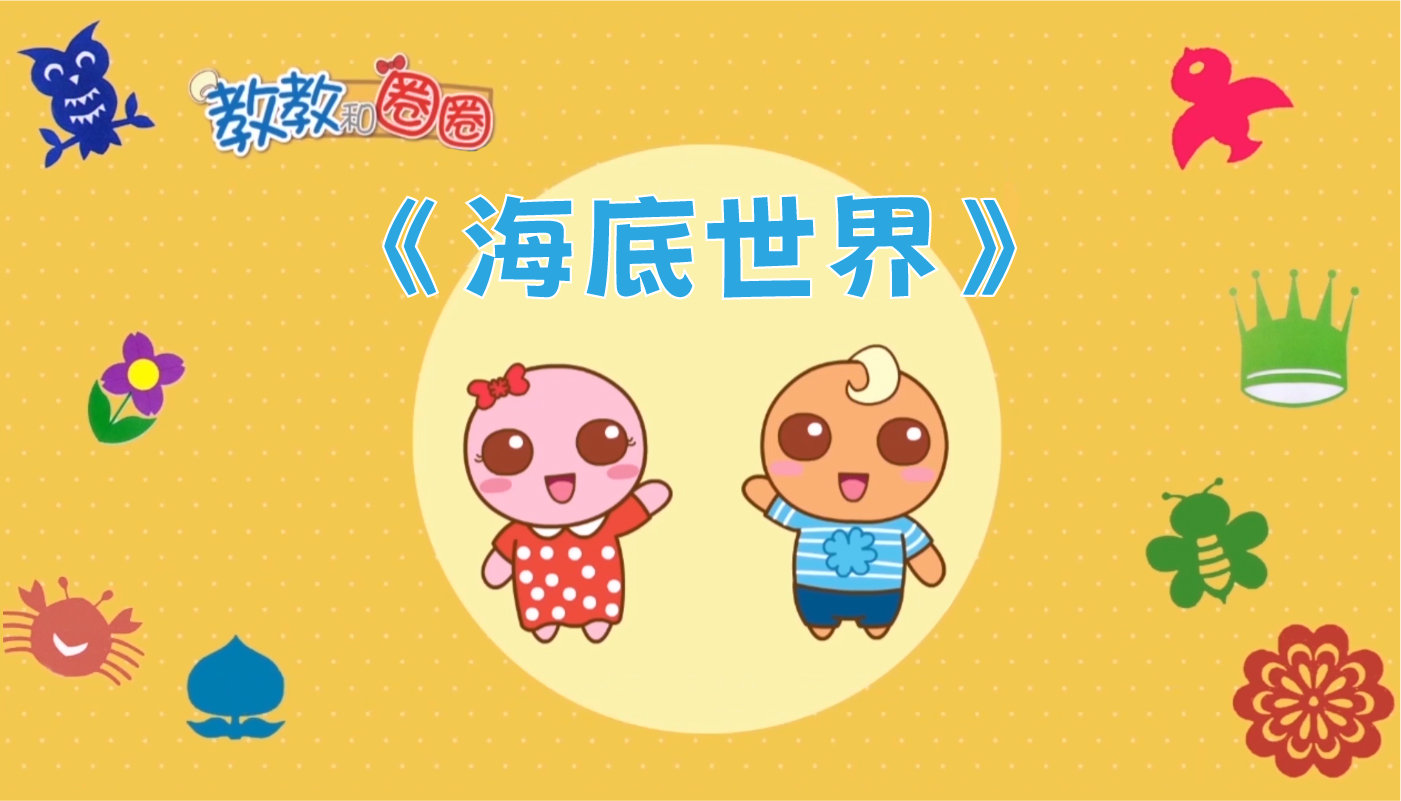海底世界
STEAM创作
•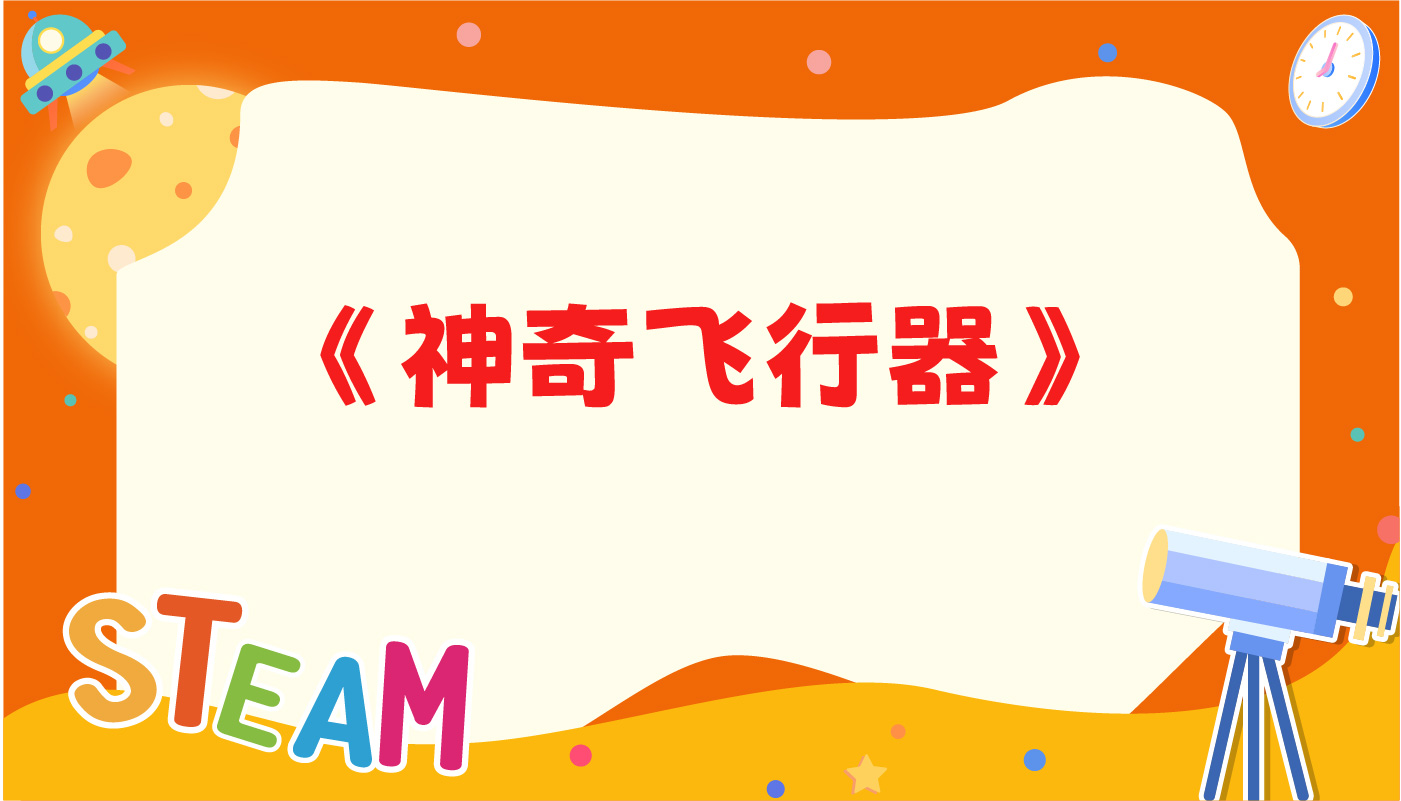神奇飞行器
•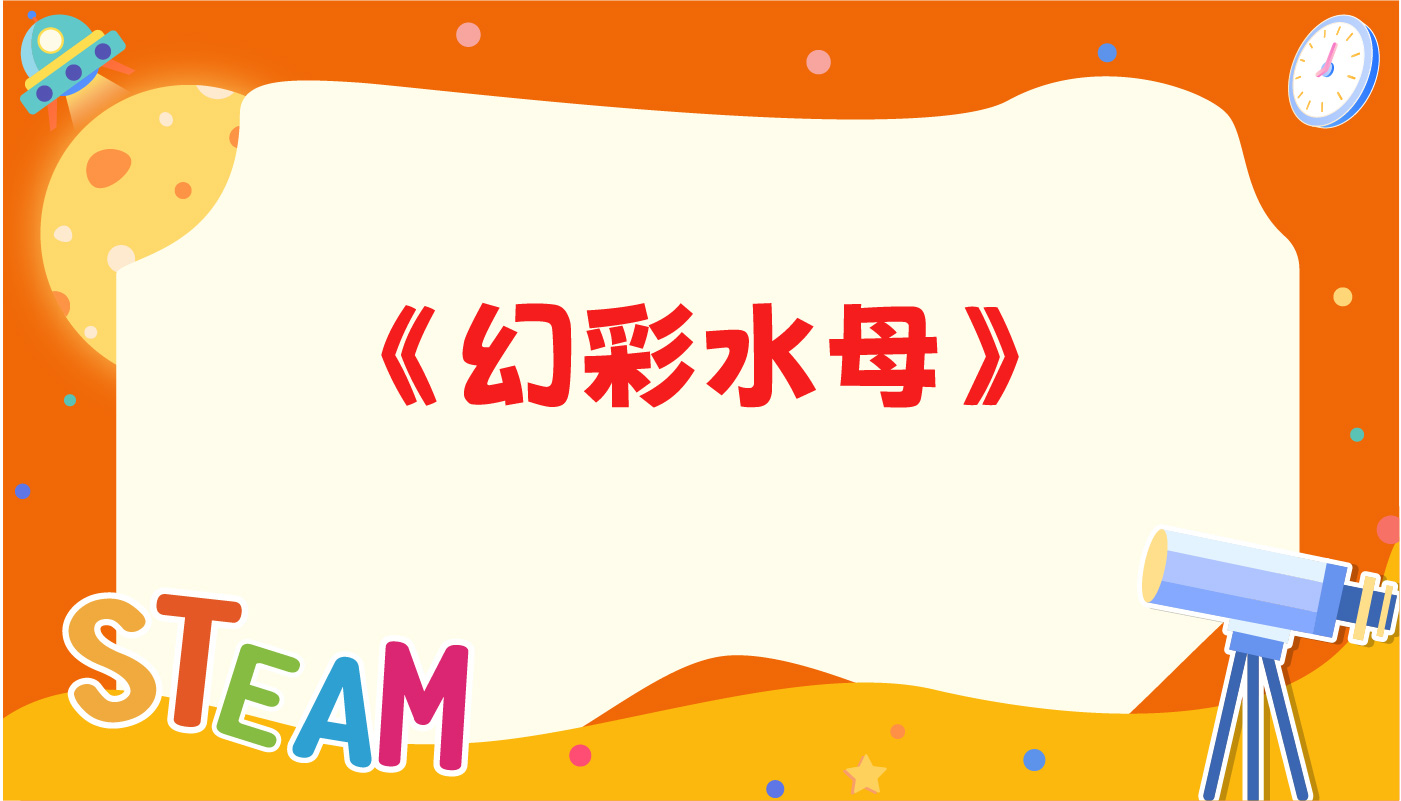幻彩水母
•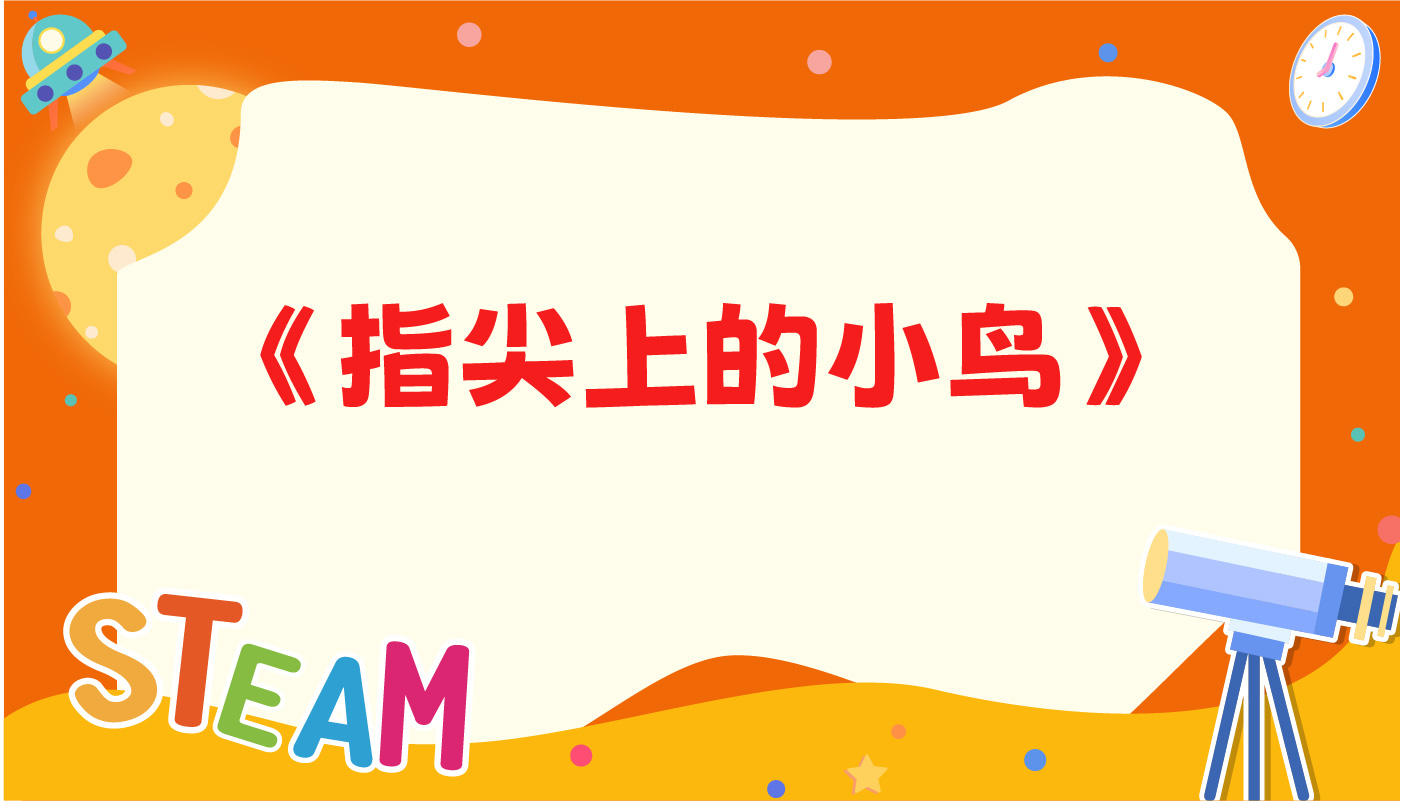指尖上的小鸟
•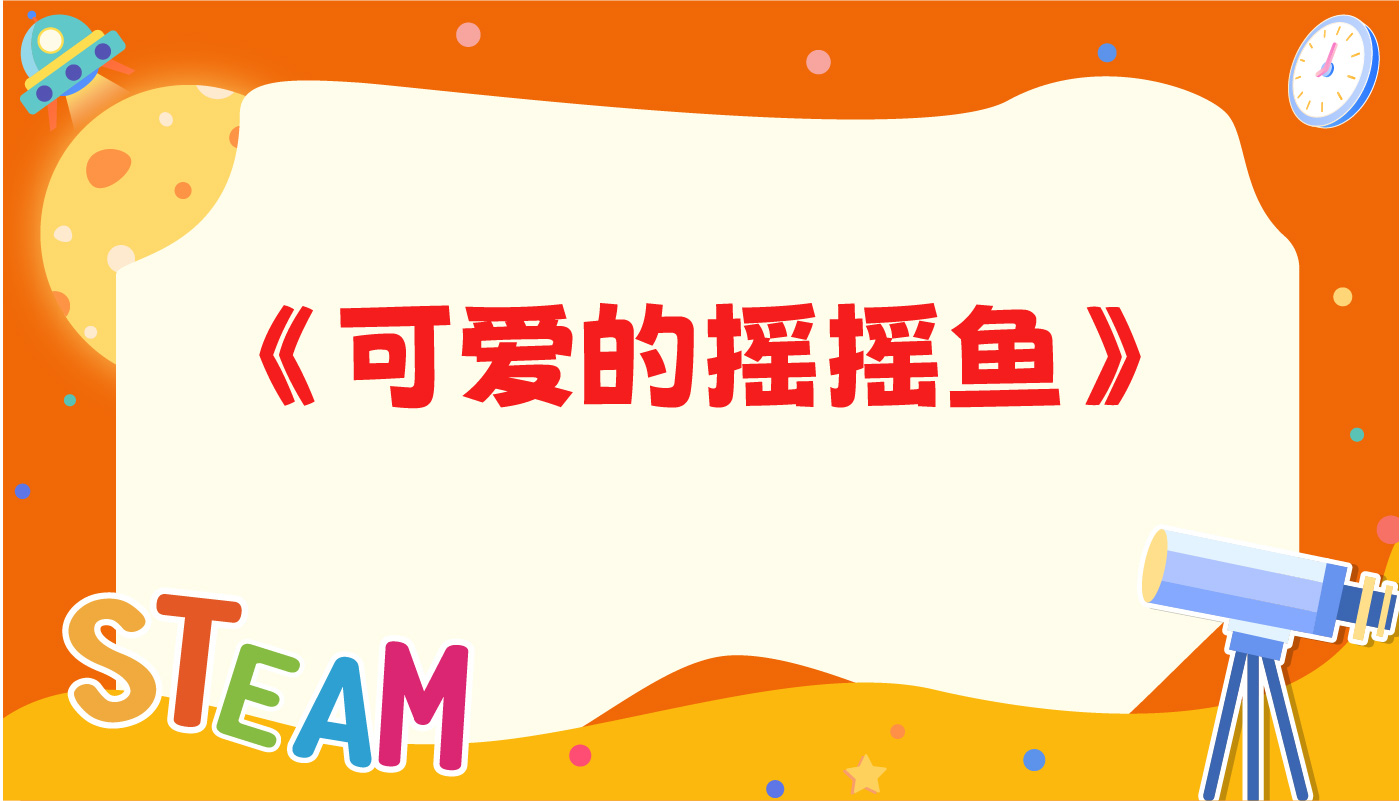可爱的摇摇鱼

•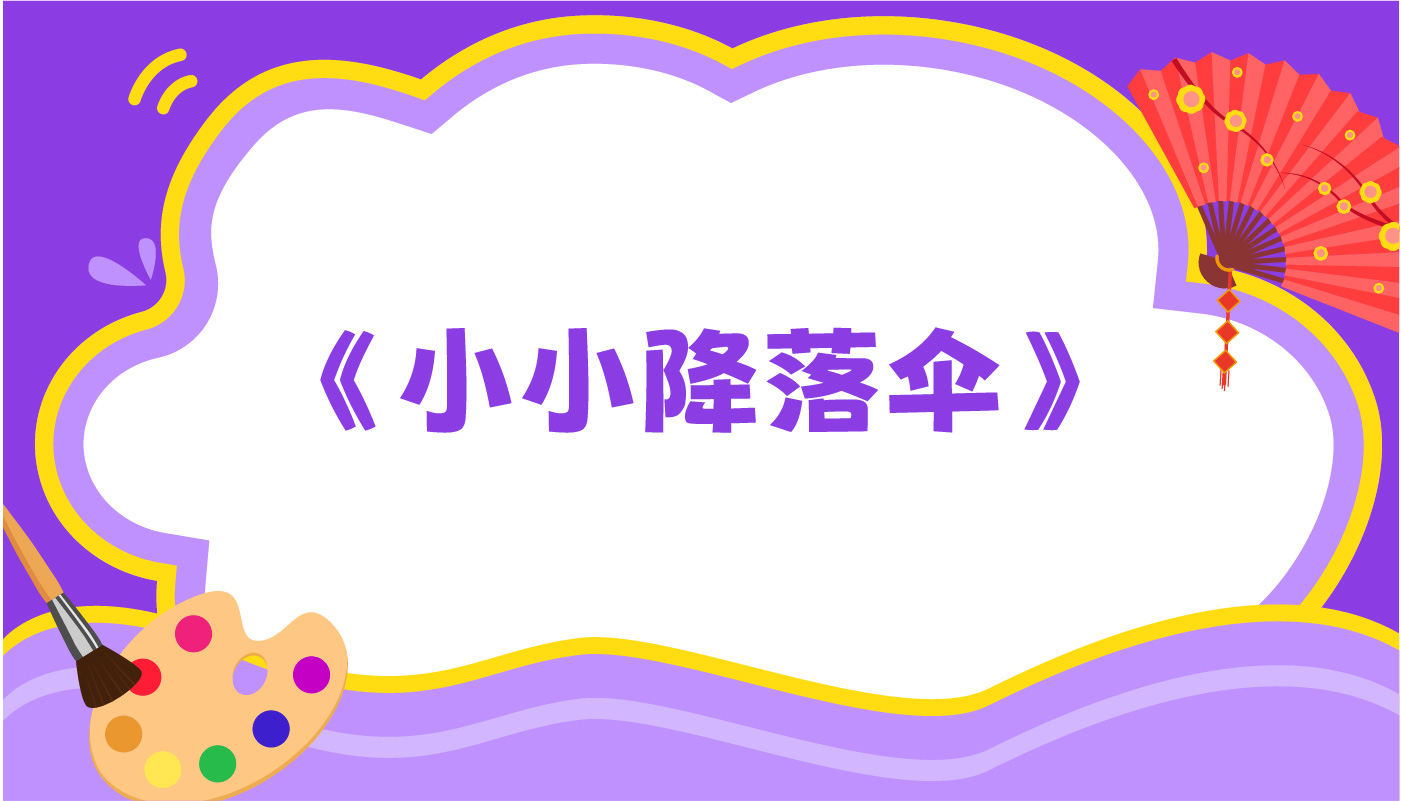小小降落伞
•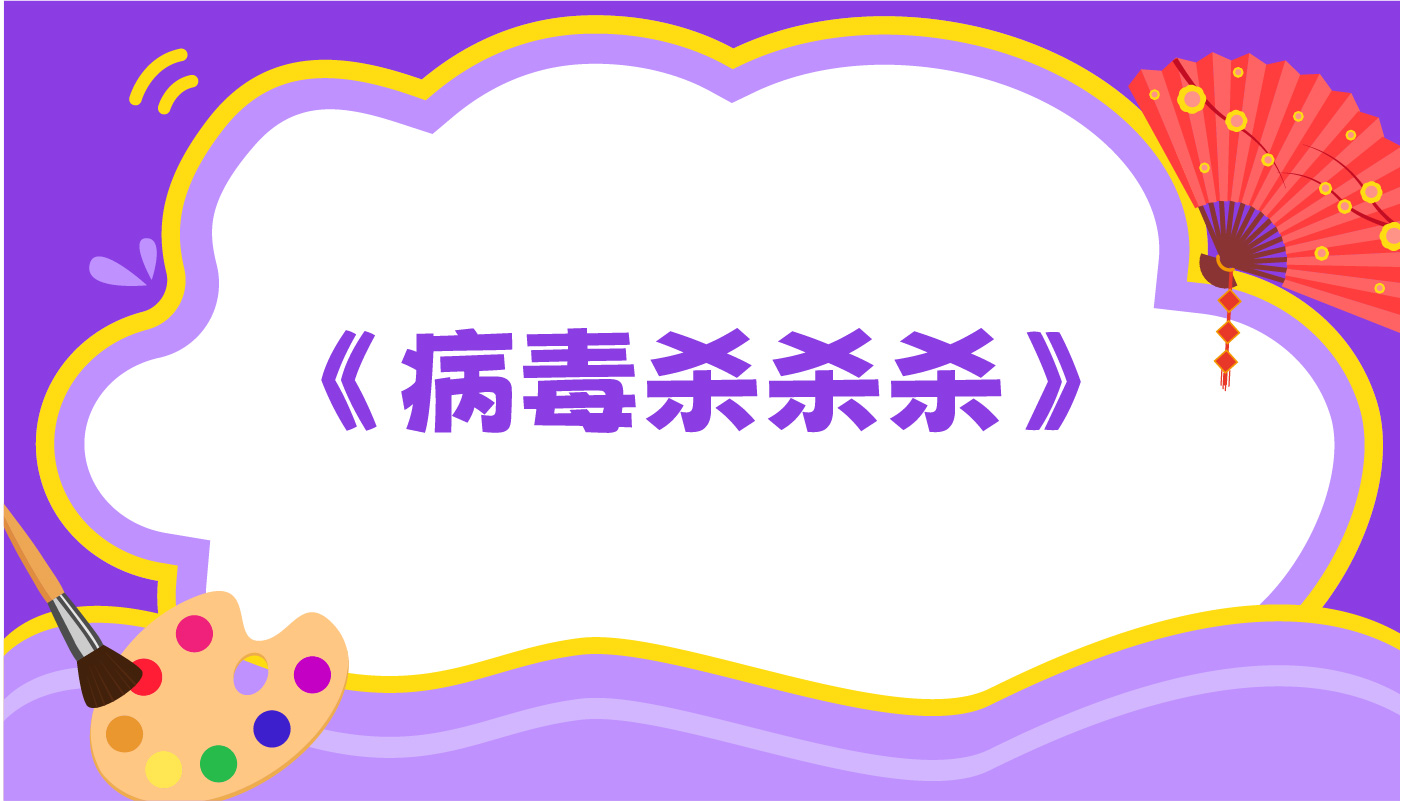病毒杀杀杀
•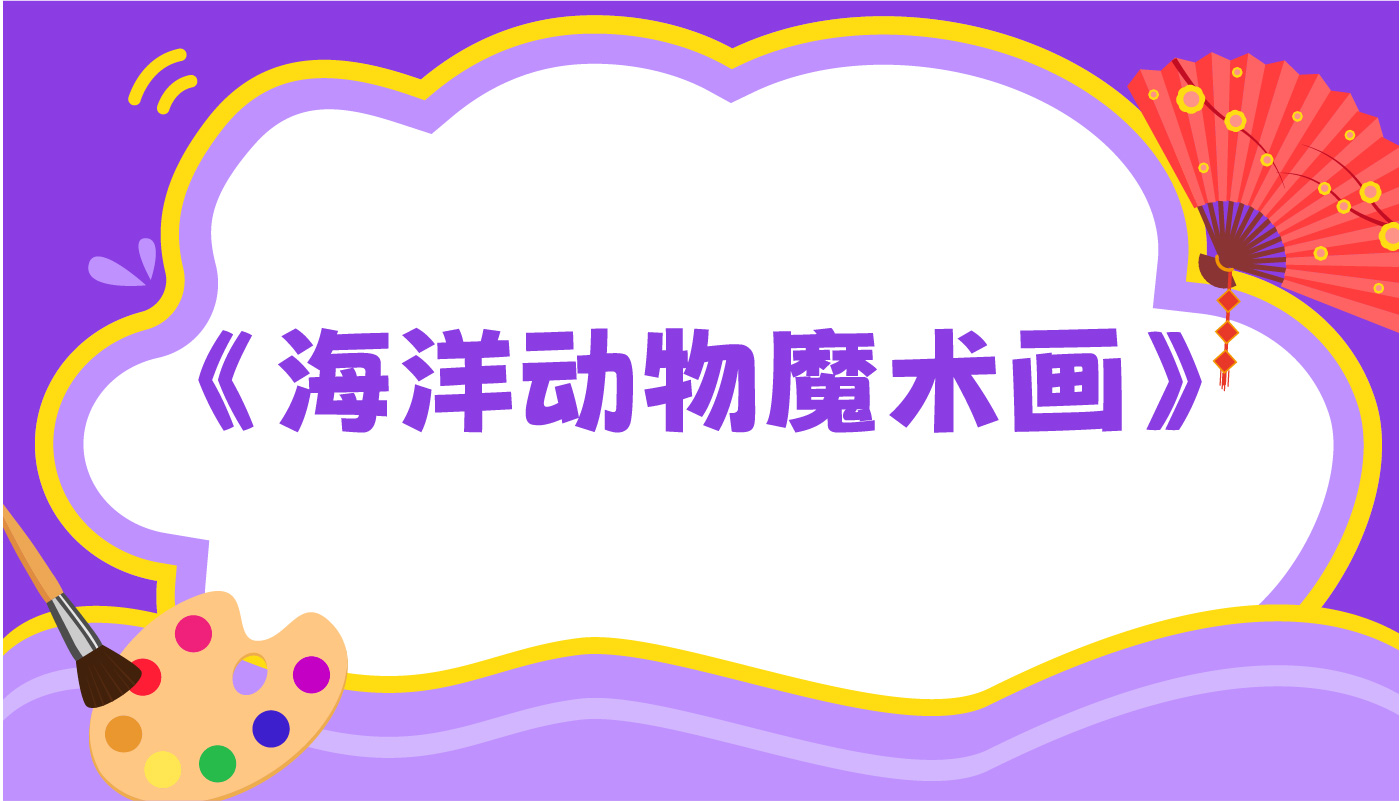海洋动物魔术画
•小老鼠上灯台
• 神奇的牙签
• 小兔跳跳
• 小老鼠上灯台
• 海洋动物魔术画
• 病毒杀杀杀
• 小小降落伞
• 小水玩球
• 蜡烛跷跷板
• 彩色的手套
• 消失的硬币
• 小黄鸡
• 神奇飞行器
• 窗花
• 藏在水里的眼睛
• 漩涡蜡烛
• 萝卜蘑菇

•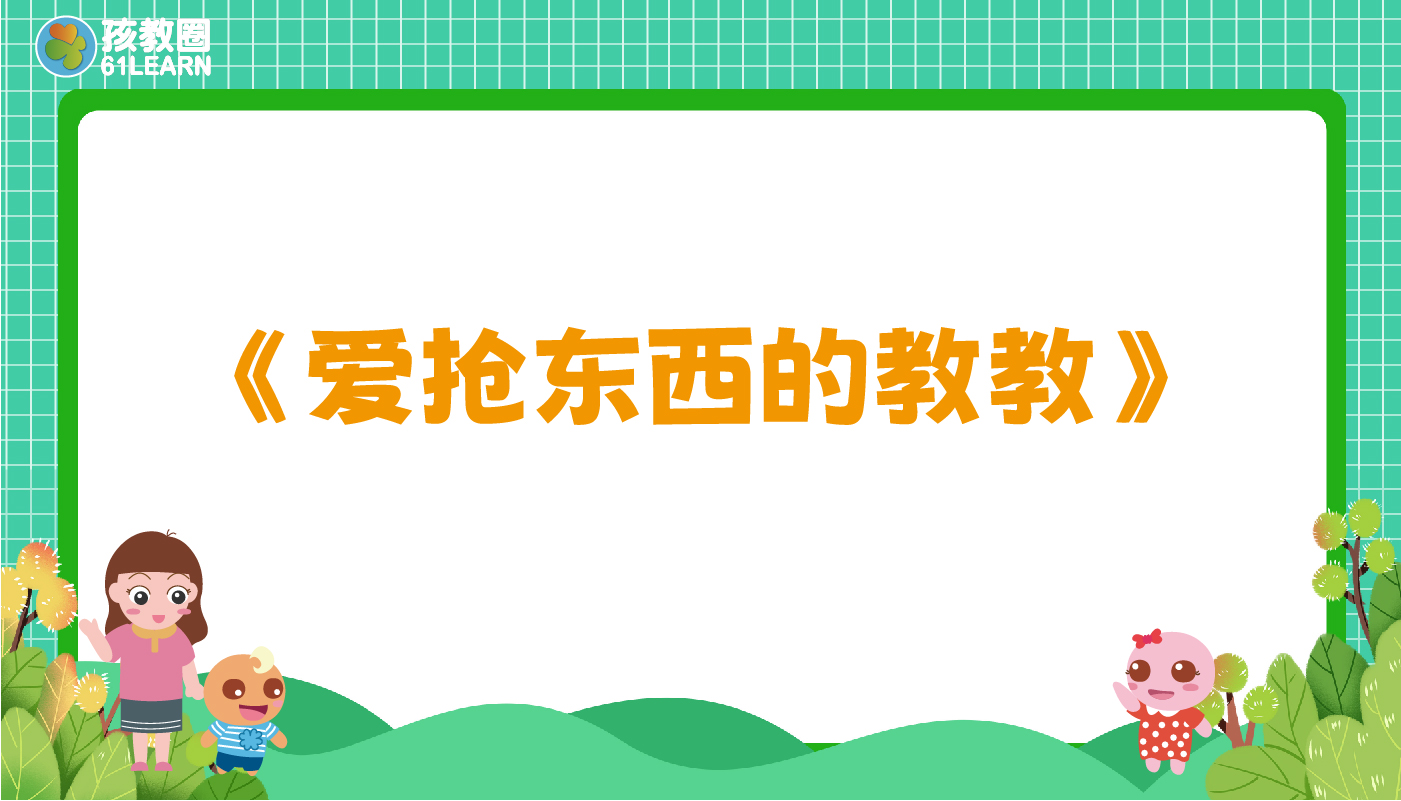爱抢东西的教教
•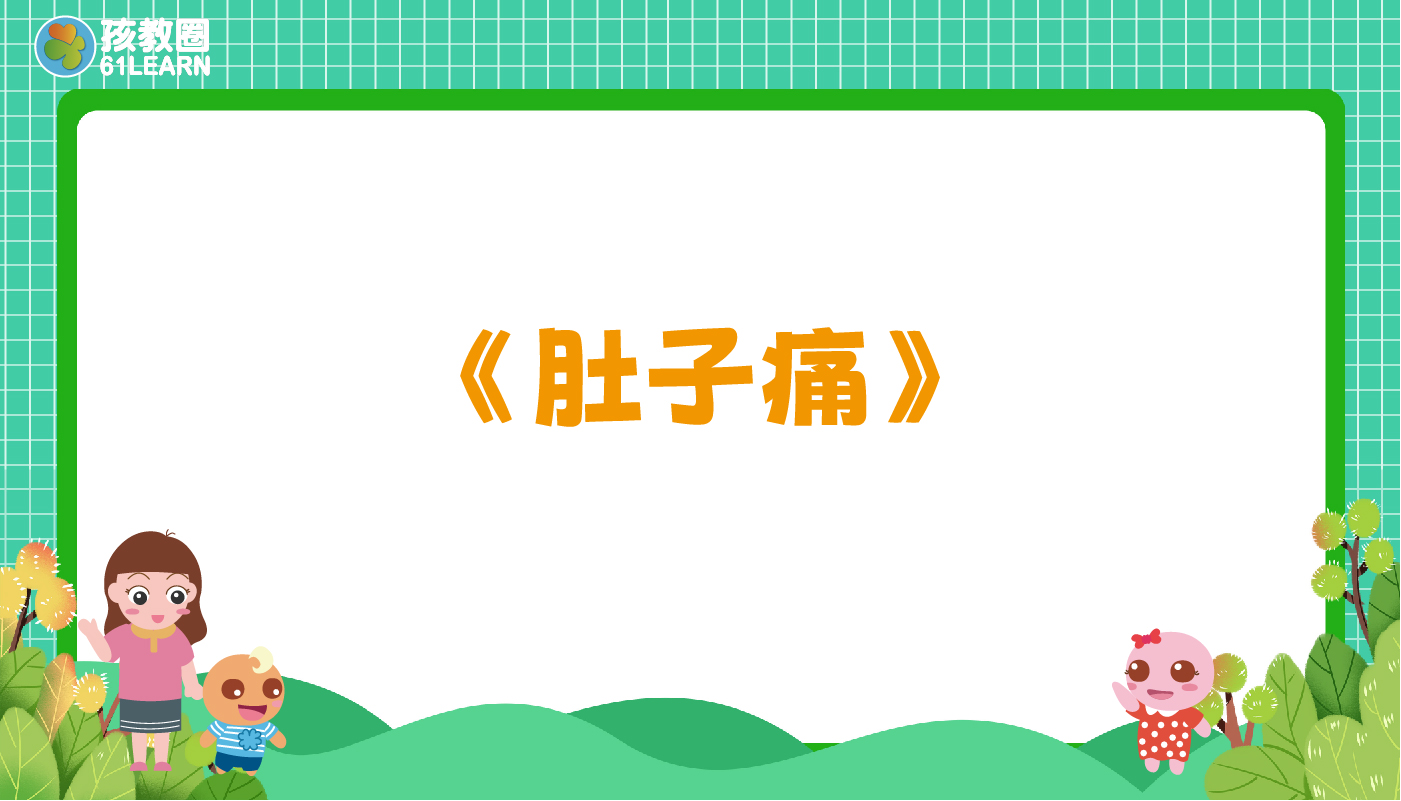肚子痛
•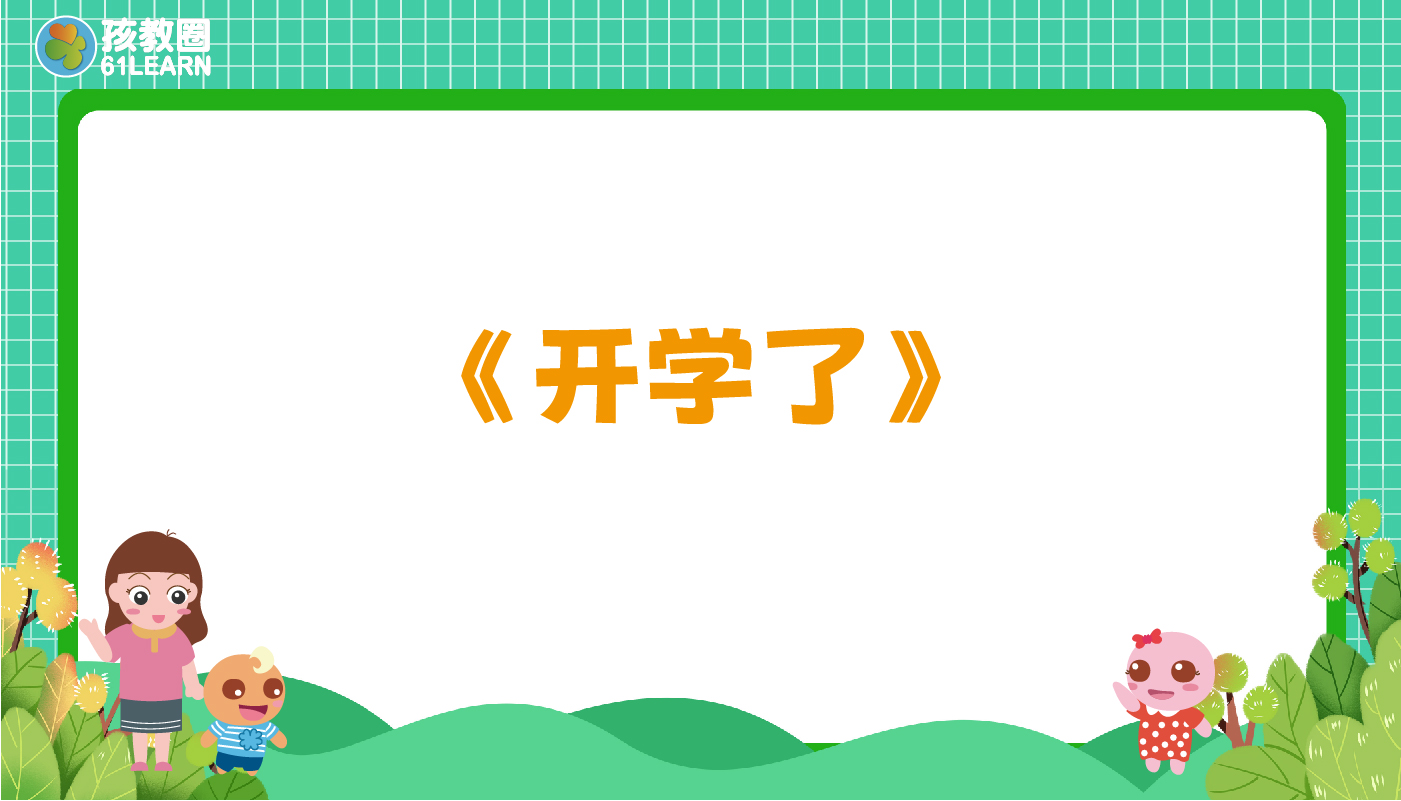开学了
•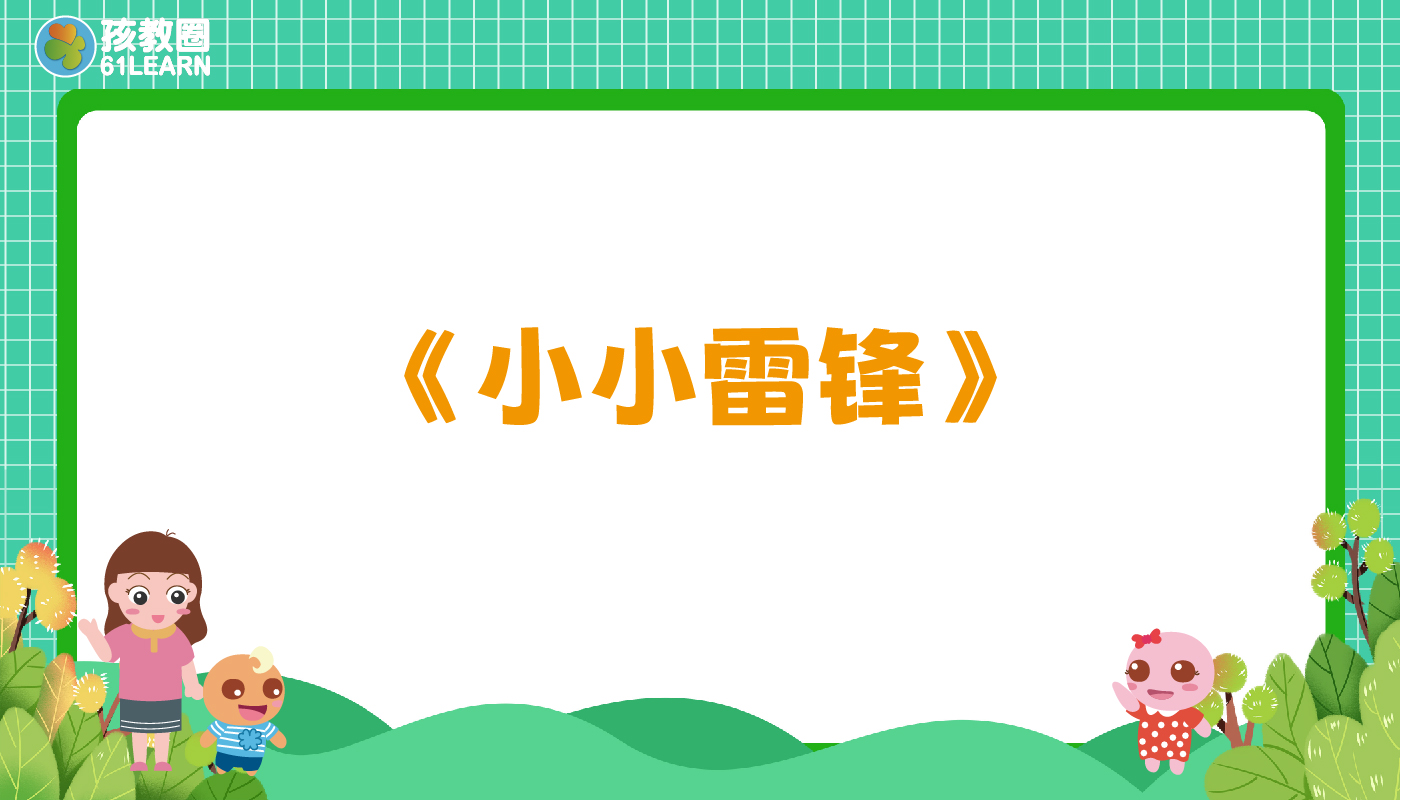小小雷锋

•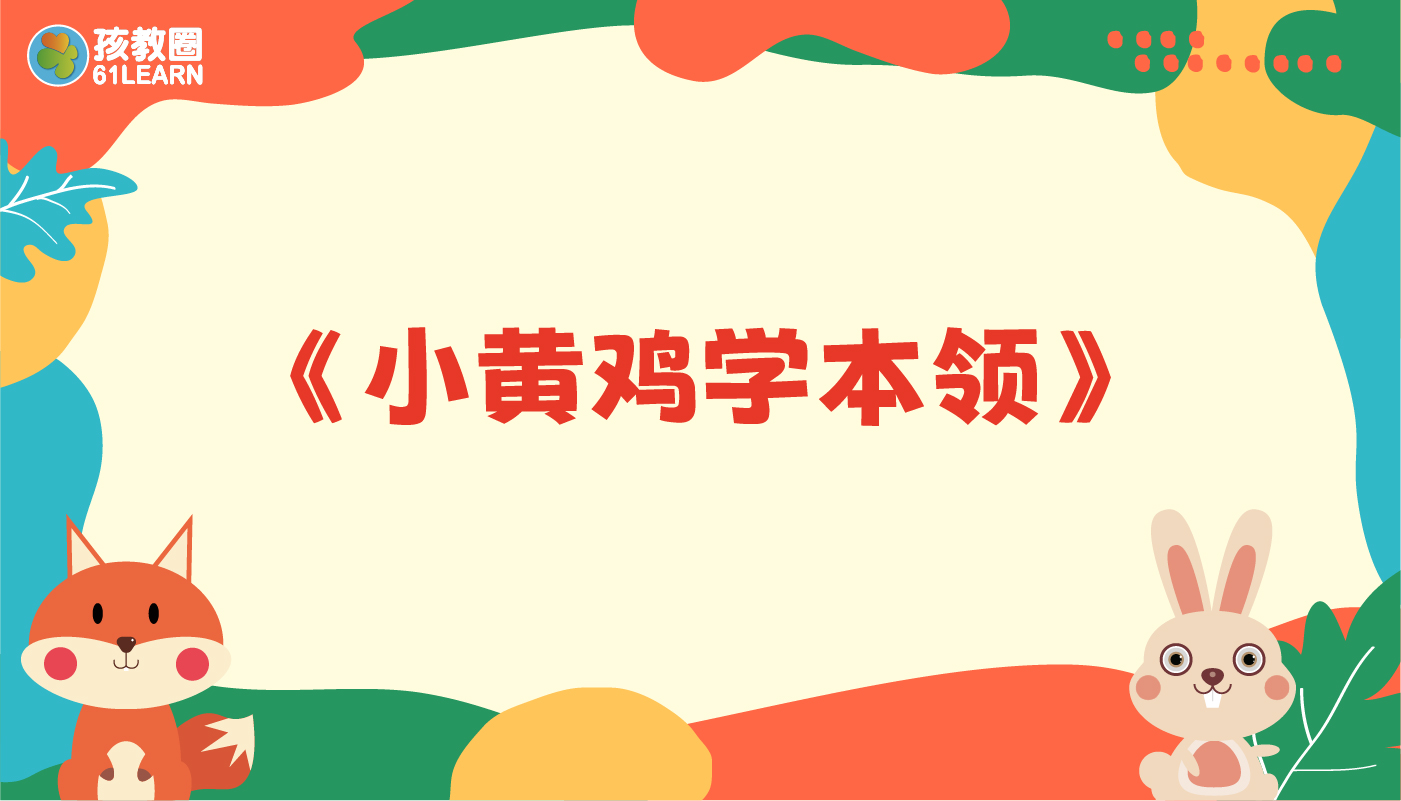小黄鸡学本领
•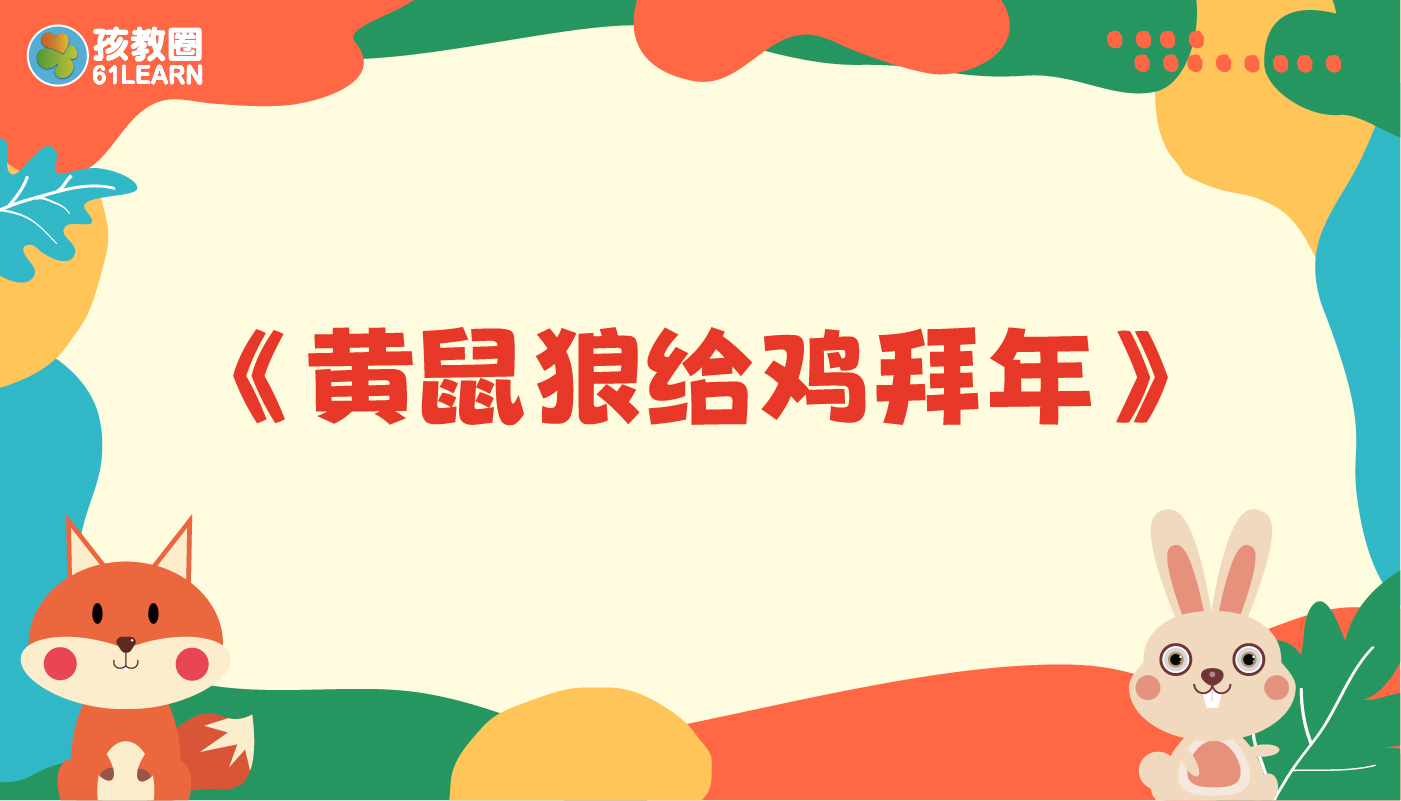黄鼠狼给鸡拜年
•勇敢的小蓝猪
•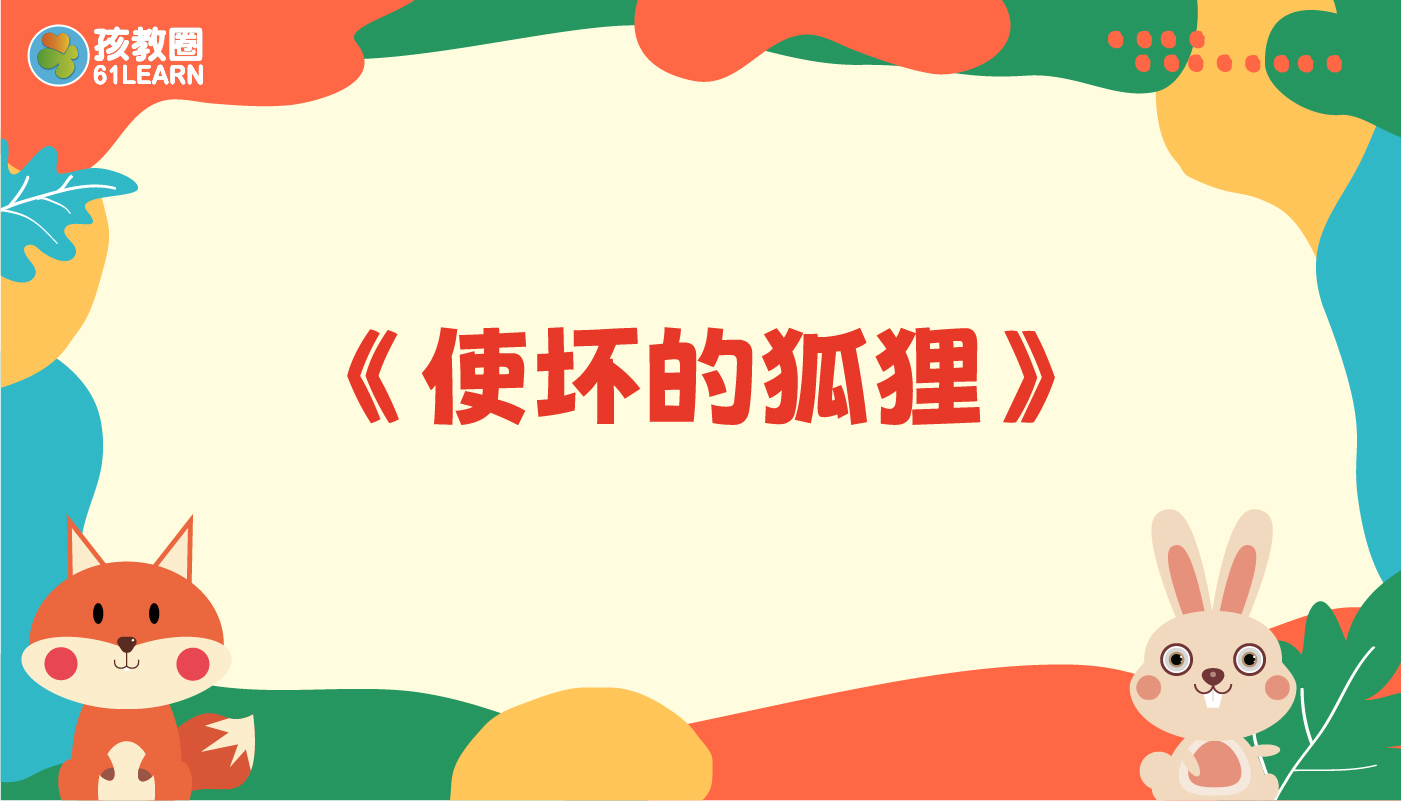使坏的狐狸

•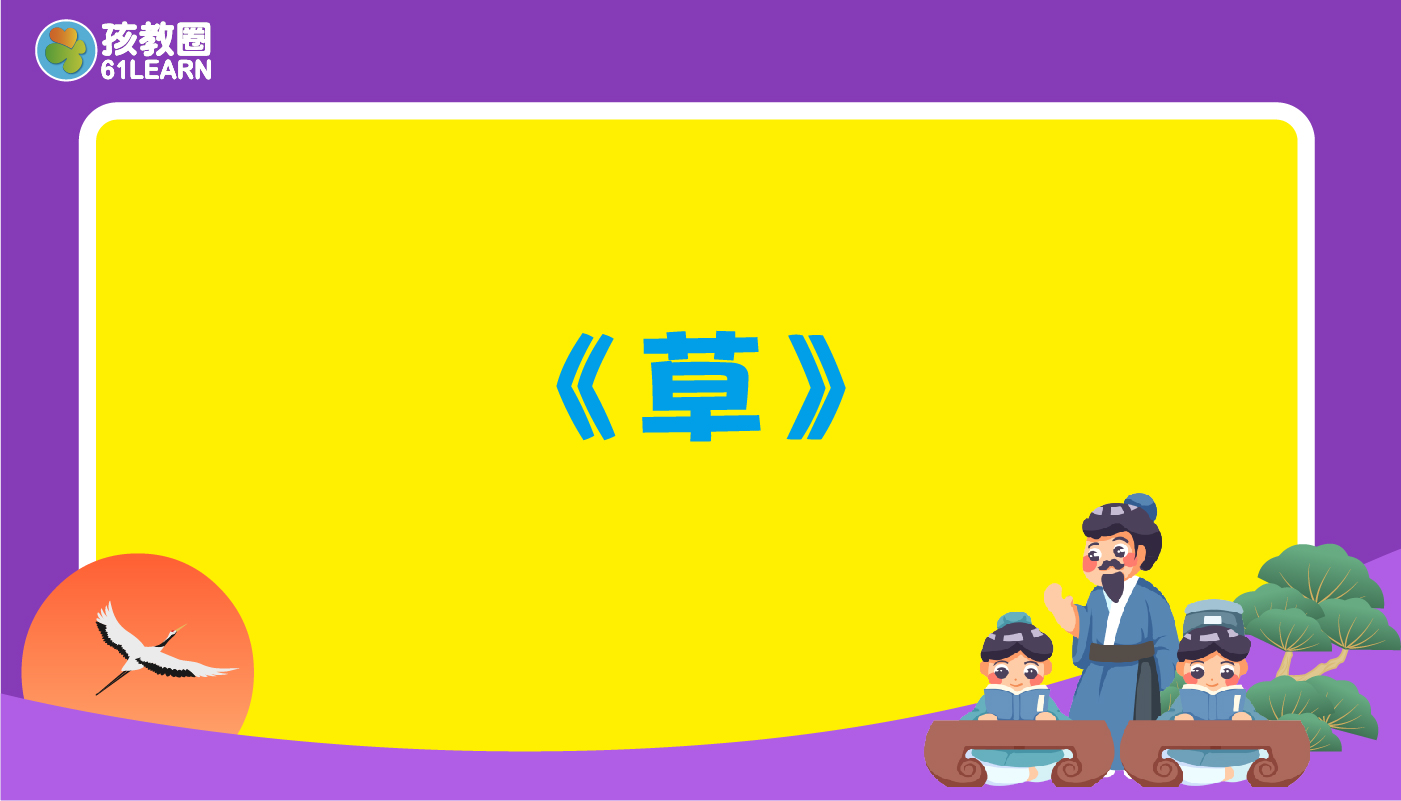•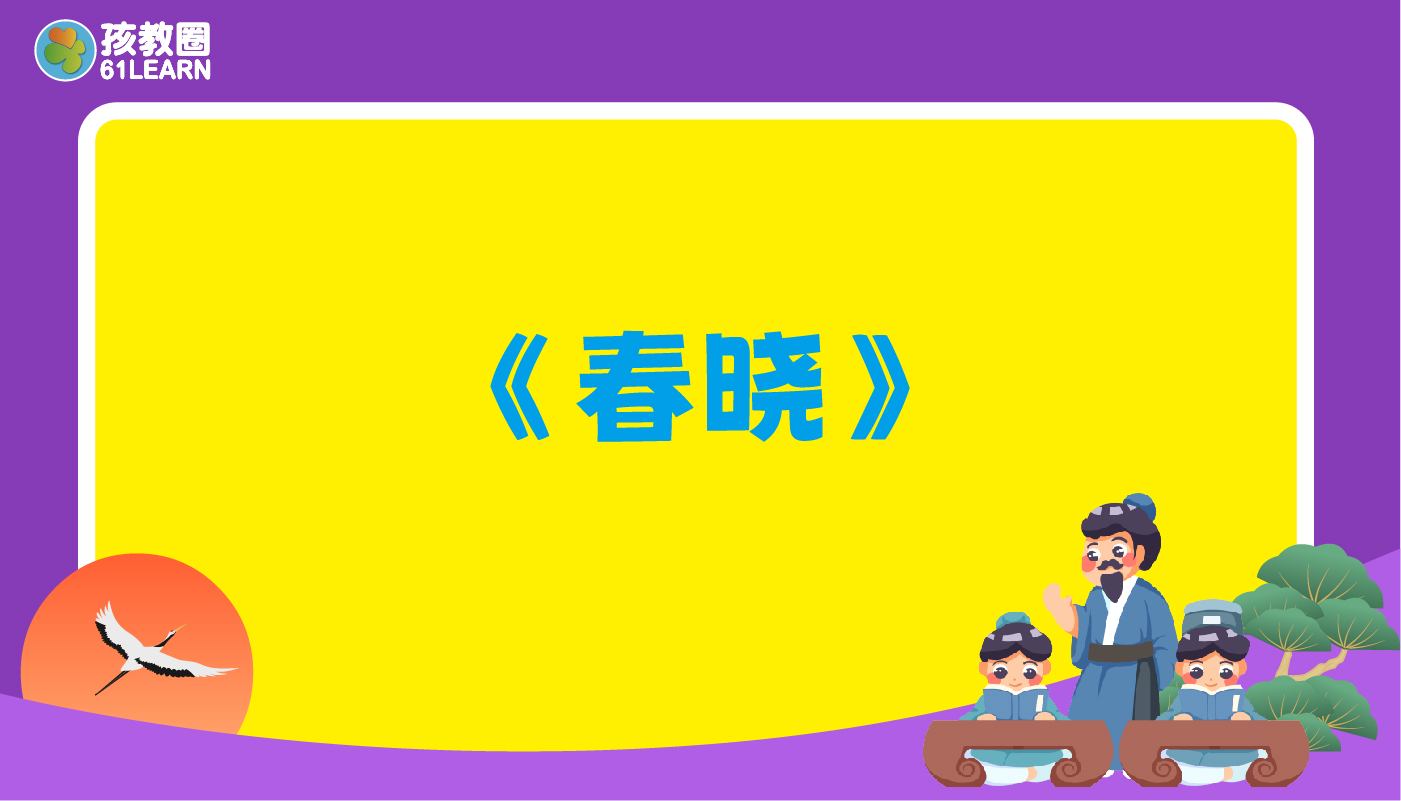春晓
•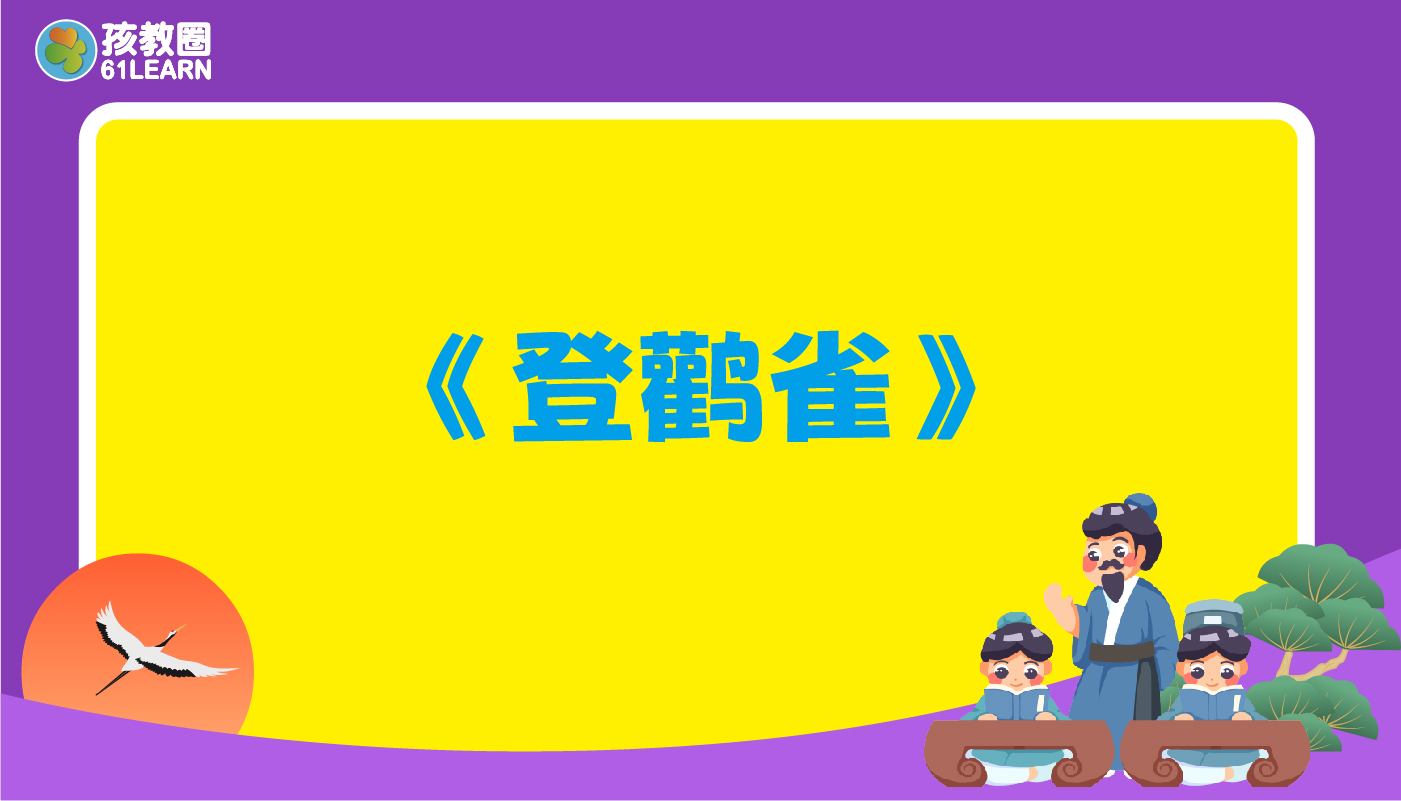登鹳雀楼
•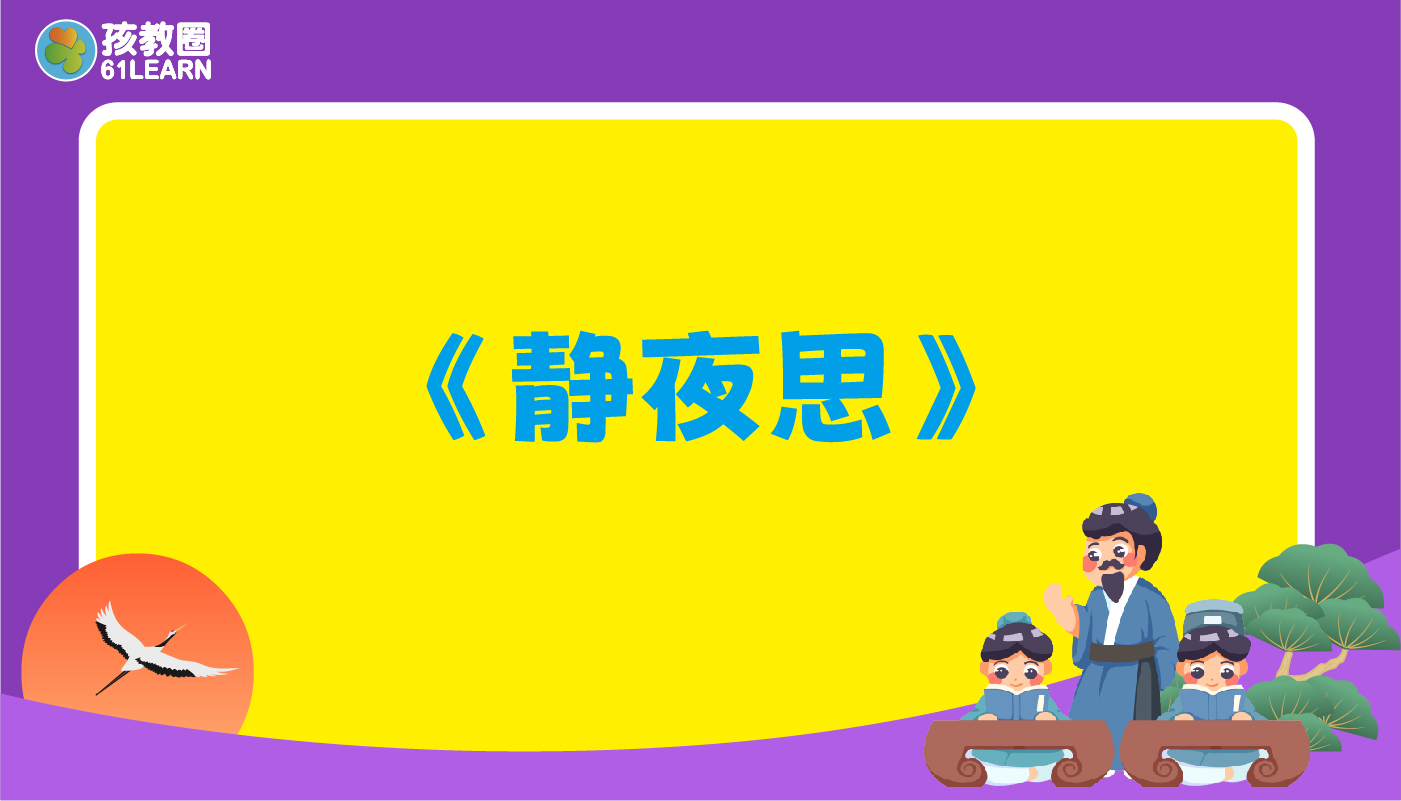静夜思

•第一节：人之初，性本善
•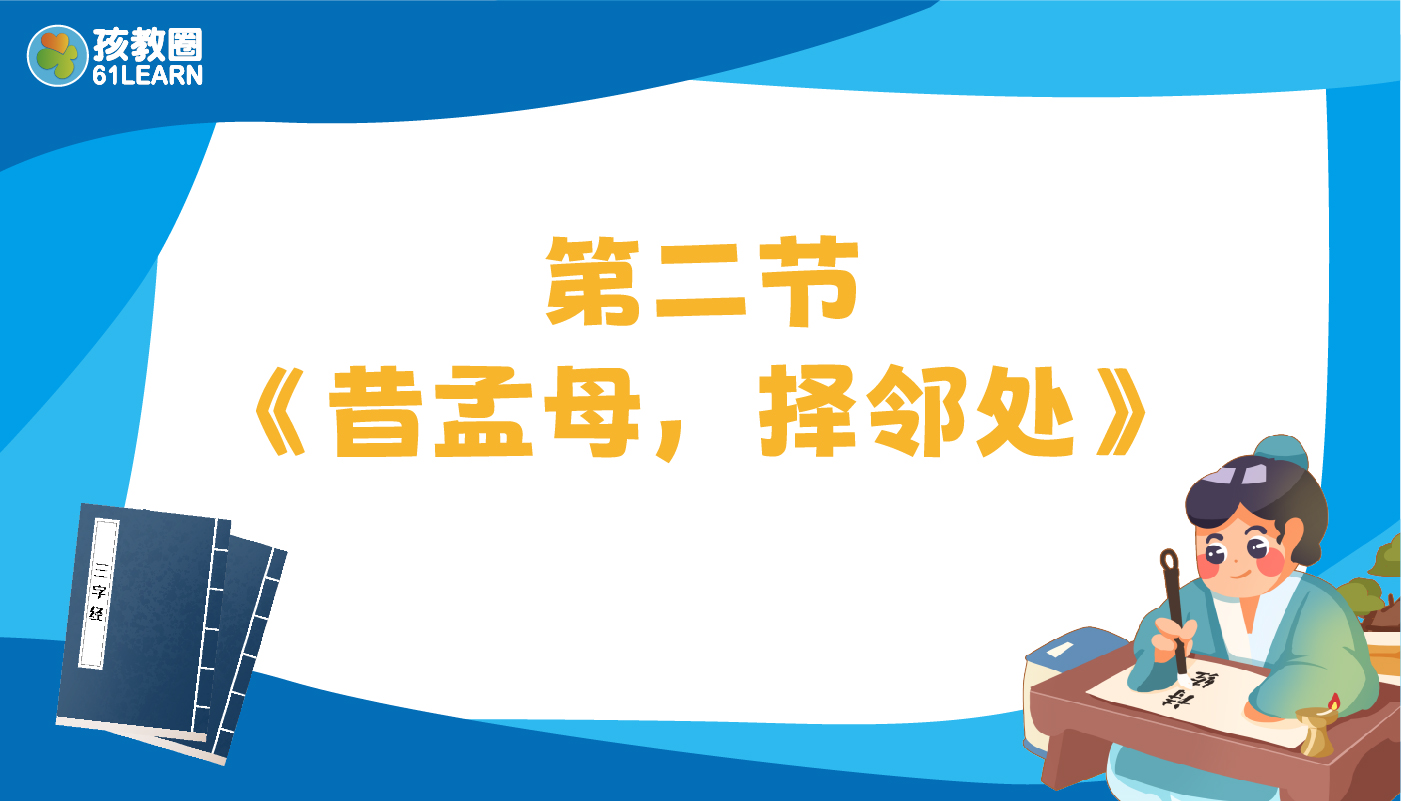第二节：昔孟母，择邻处
•第三节：养不教，父之过
•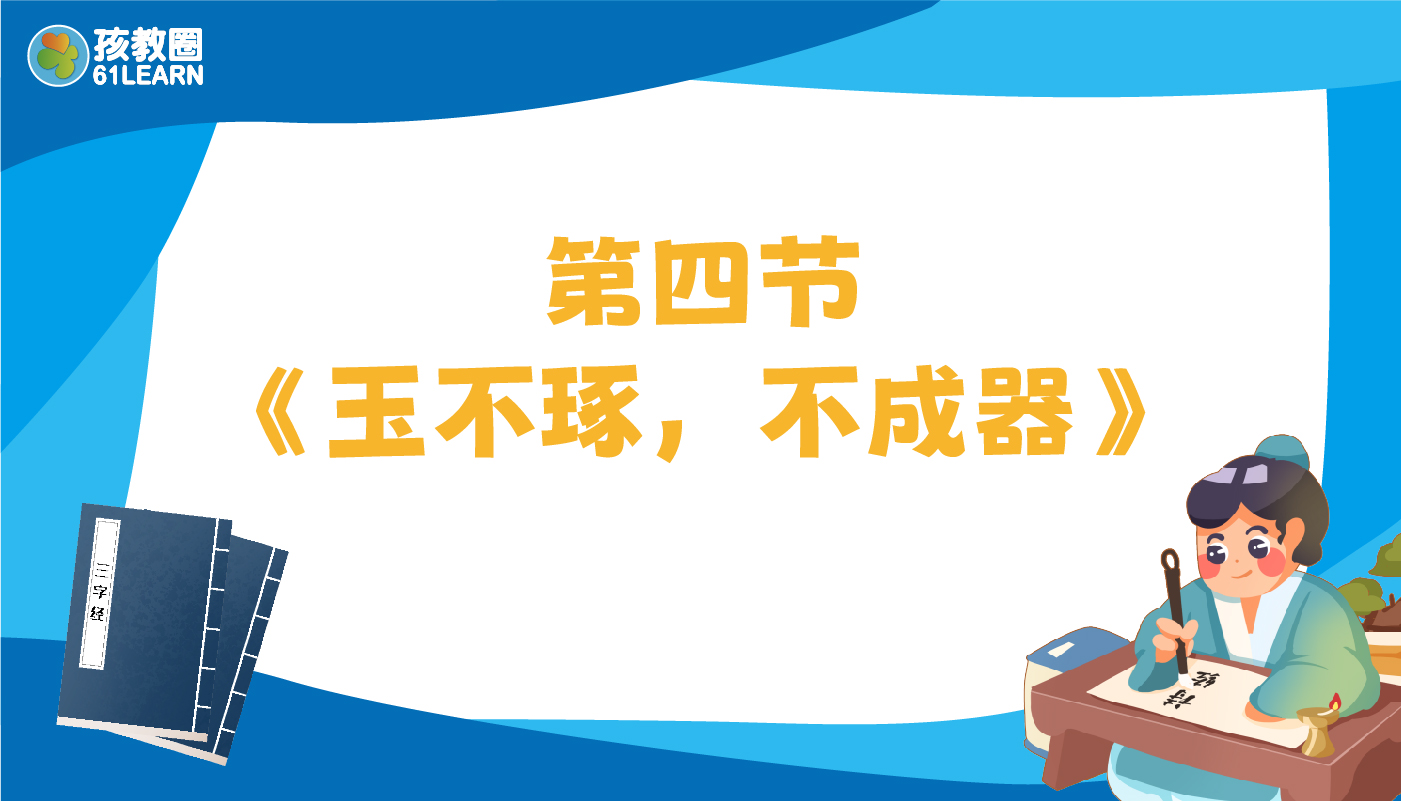第四节：玉不琢，不成器
• 使坏的狐狸
• 第一节：人之初，性本善
• 第五节：香九龄，能温席
• 第四节：玉不琢，不成器
• 第三节：养不教，父之过
• 第二节：昔孟母，择邻处
• 静夜思
• 登鹳雀楼
• 爱抢东西的教教
• 小黄鸡学本领
• 第一节：人之初，性本善
• 第二节：昔孟母，择邻处
• 肚子痛
• 黄鼠狼给鸡拜年
• 春晓